# R S Aggarwal Solutions for Class 11 Maths Chapter 17- Trigonometric Equations

R S Aggarwal Solutions are useful for students as it helps them to score well in the class 11 exams. The subject experts at BYJU’S have devised detailed chapter wise solutions for the students to understand the concepts easily. The R S Aggarwal Solutions contain detailed explanation of all the problems that come under the 17th chapter, Trigonometric Equations, of the class 11 R S Aggarwal Textbook.

By solving questions from the R S Aggarwal publication for Class 11, students will be able to understand all the concepts covered in the chapter “Trigonometric Equations.” Students can download the PDF of R S Aggarwal Solutions for Class 11 Maths Chapter 17- Trigonometric Equations from the link given below.

### Download PDF of R S Aggarwal Solutions for Class 11 Maths Chapter 17- Trigonometric Equations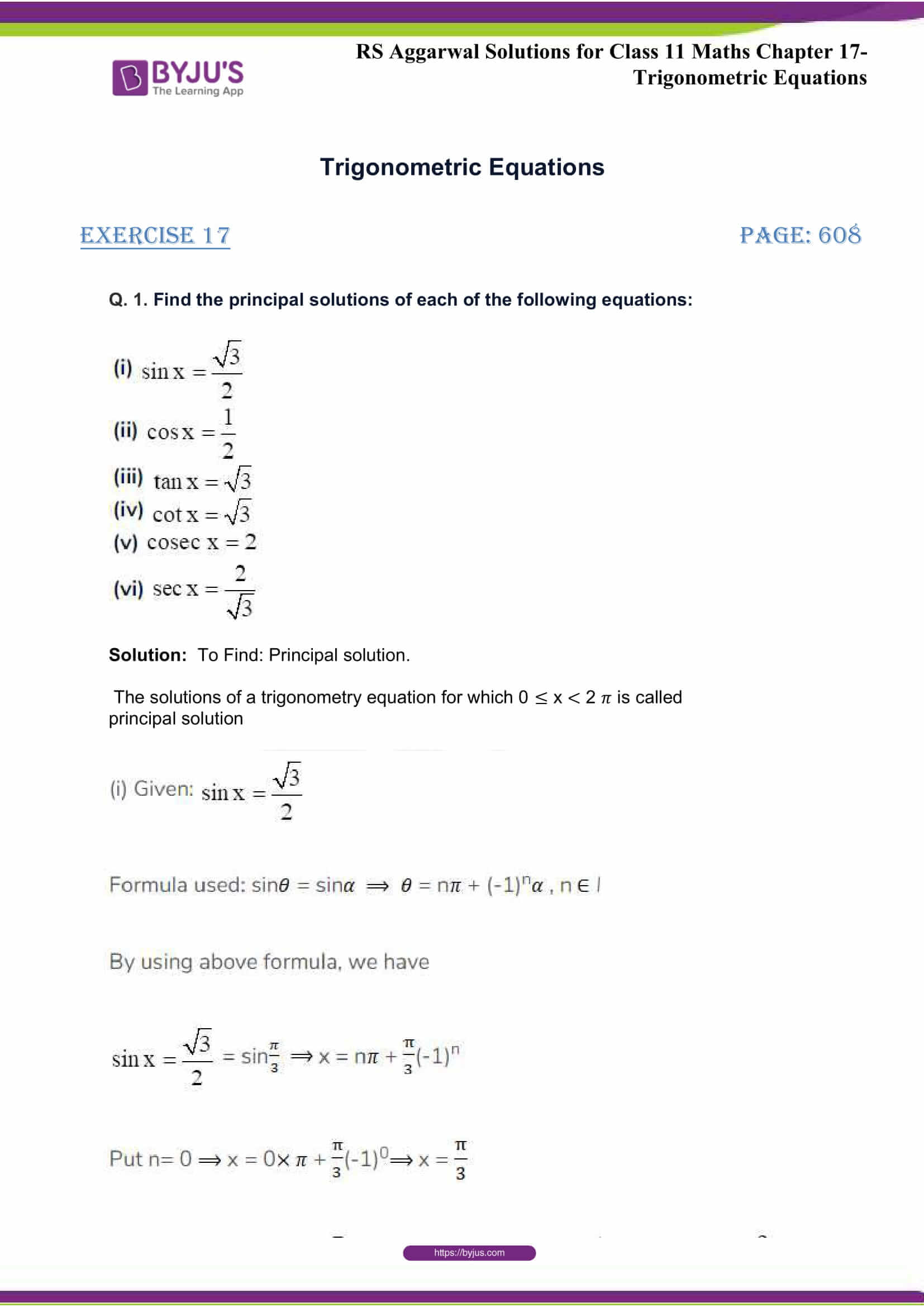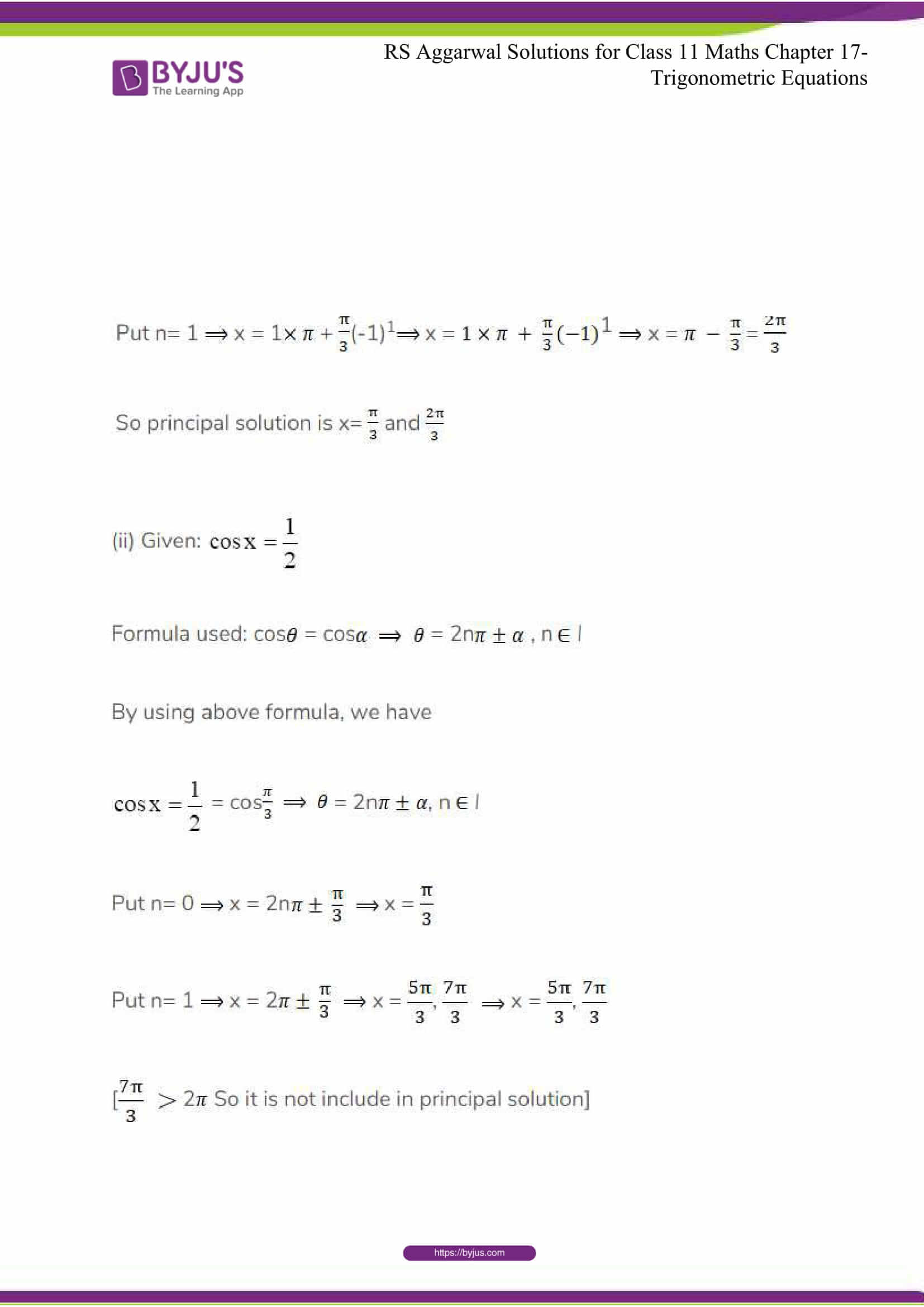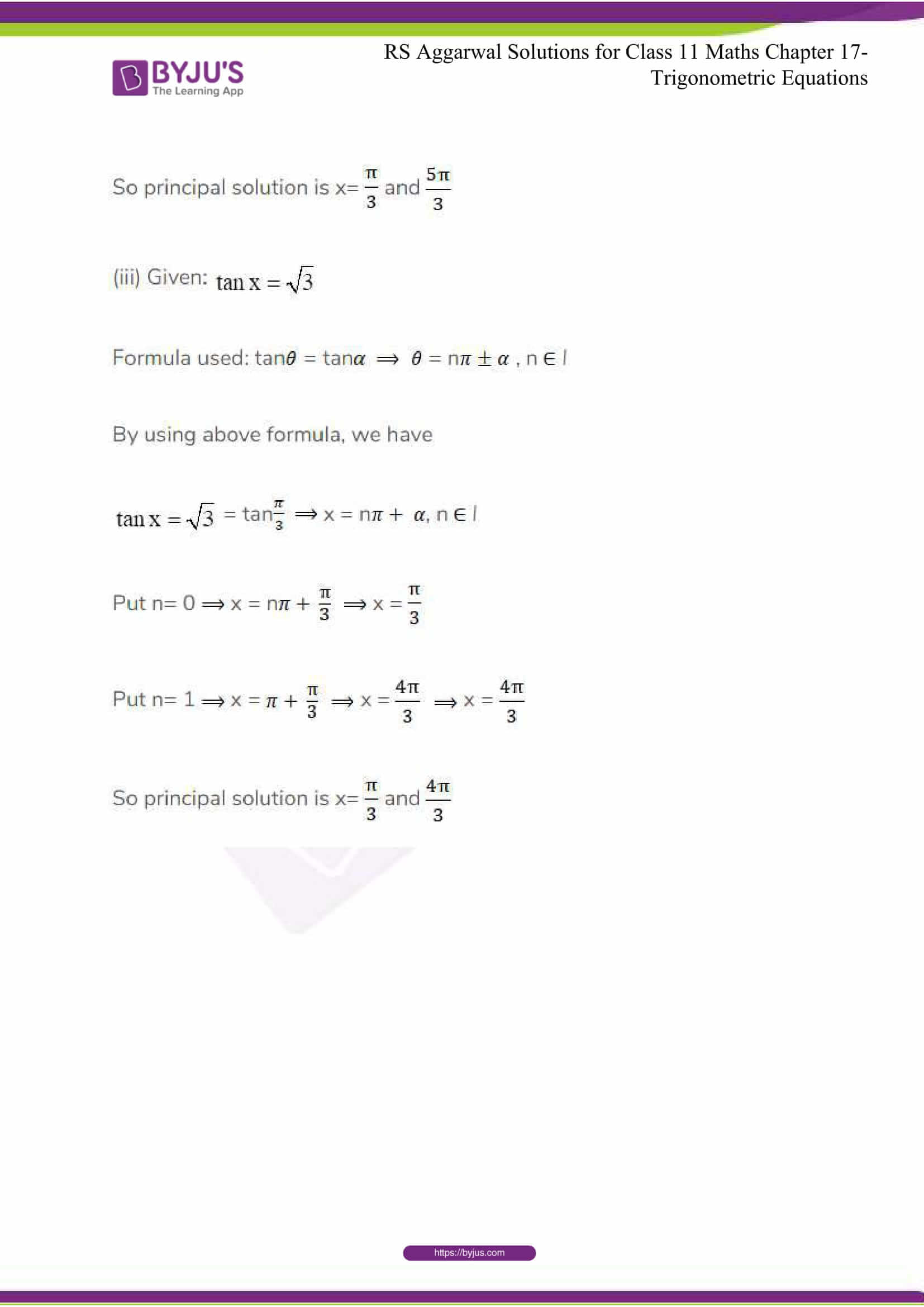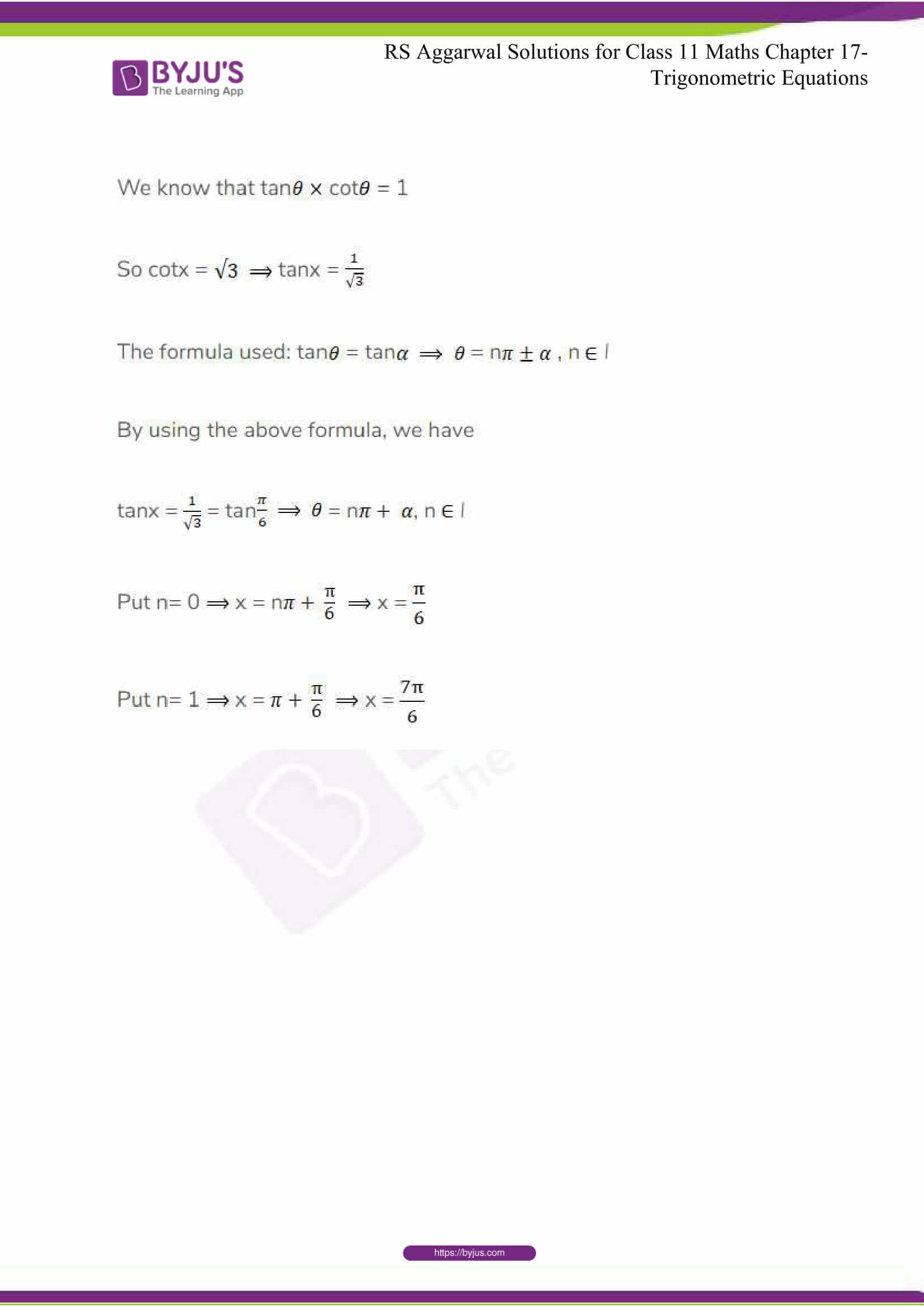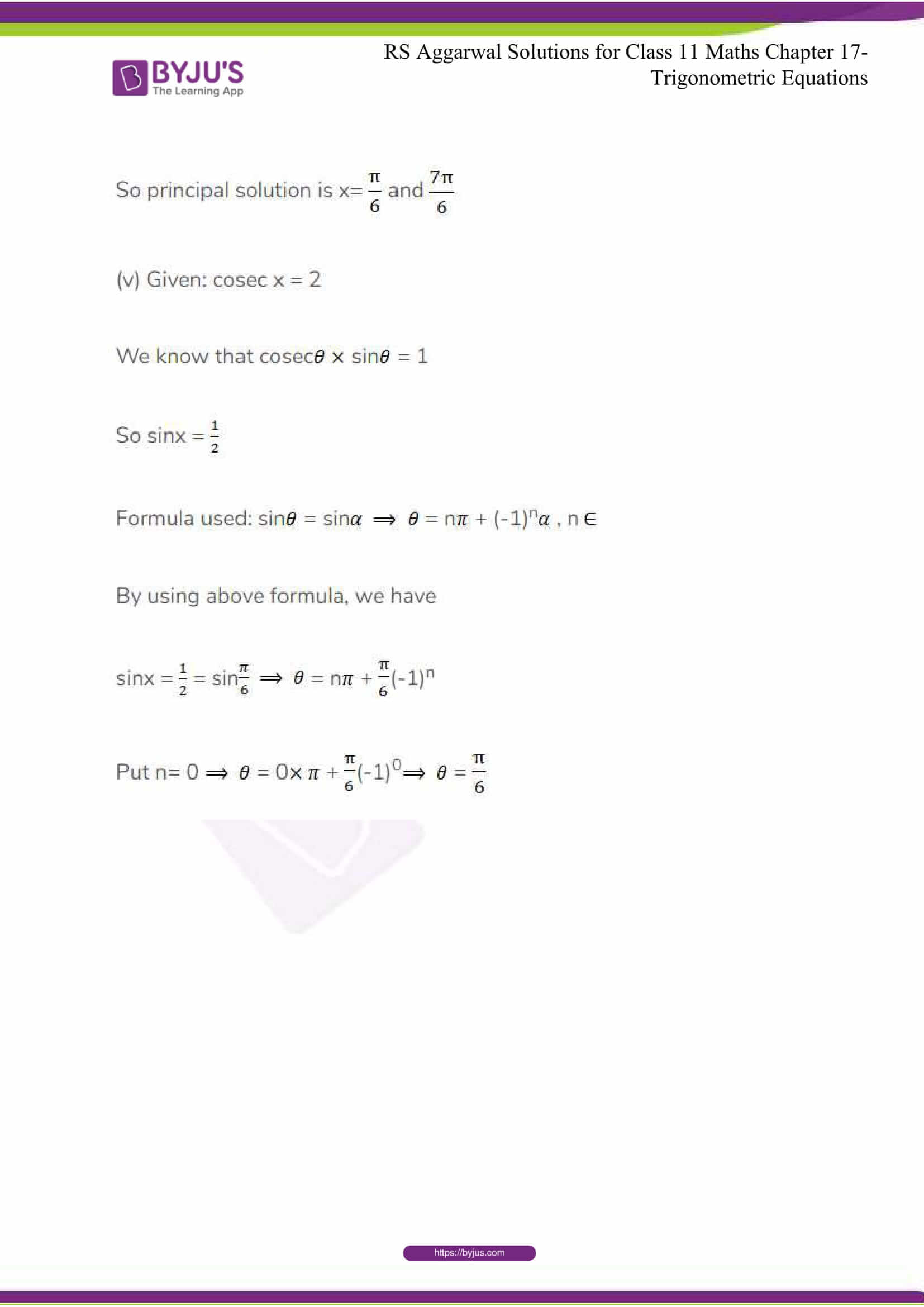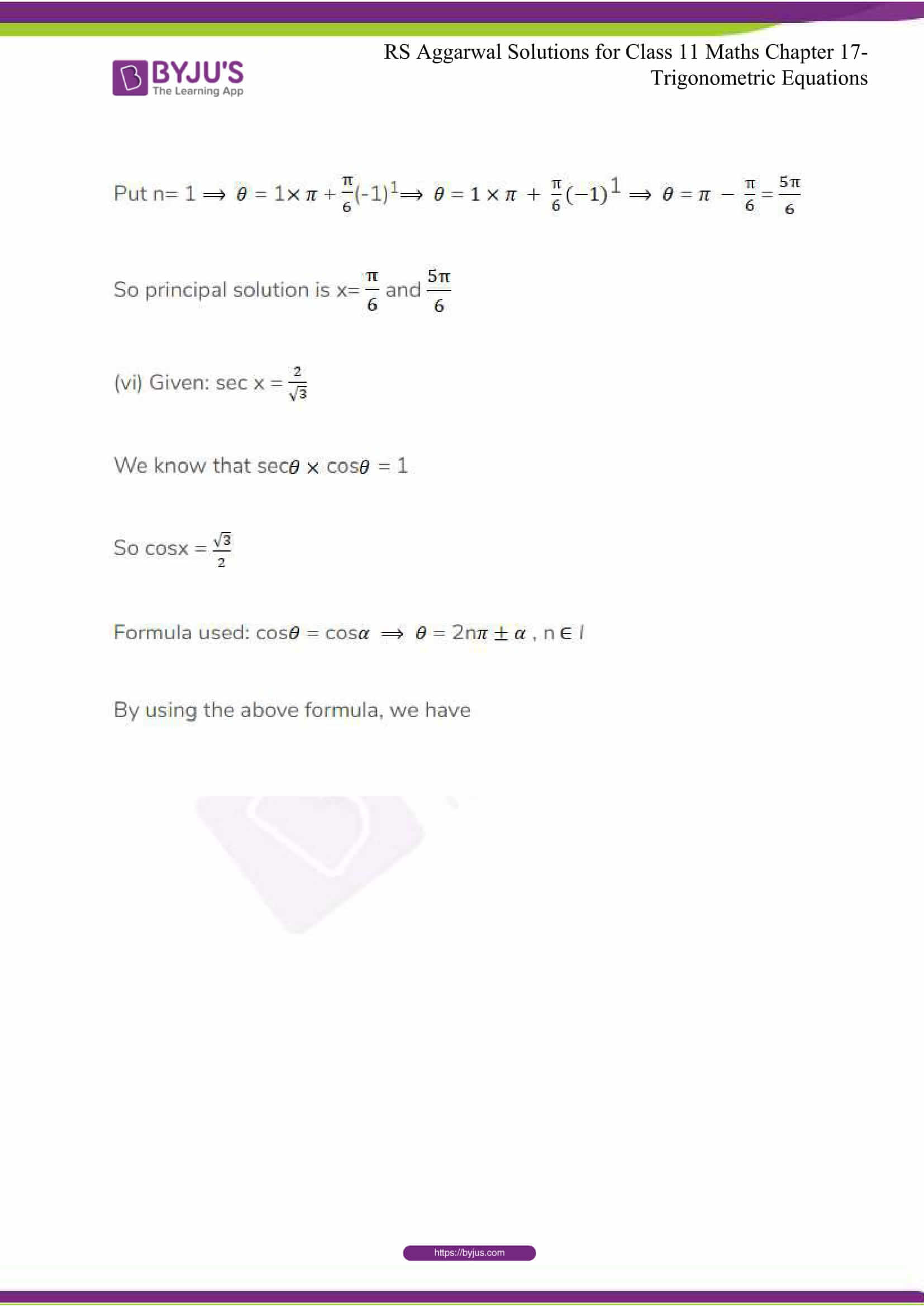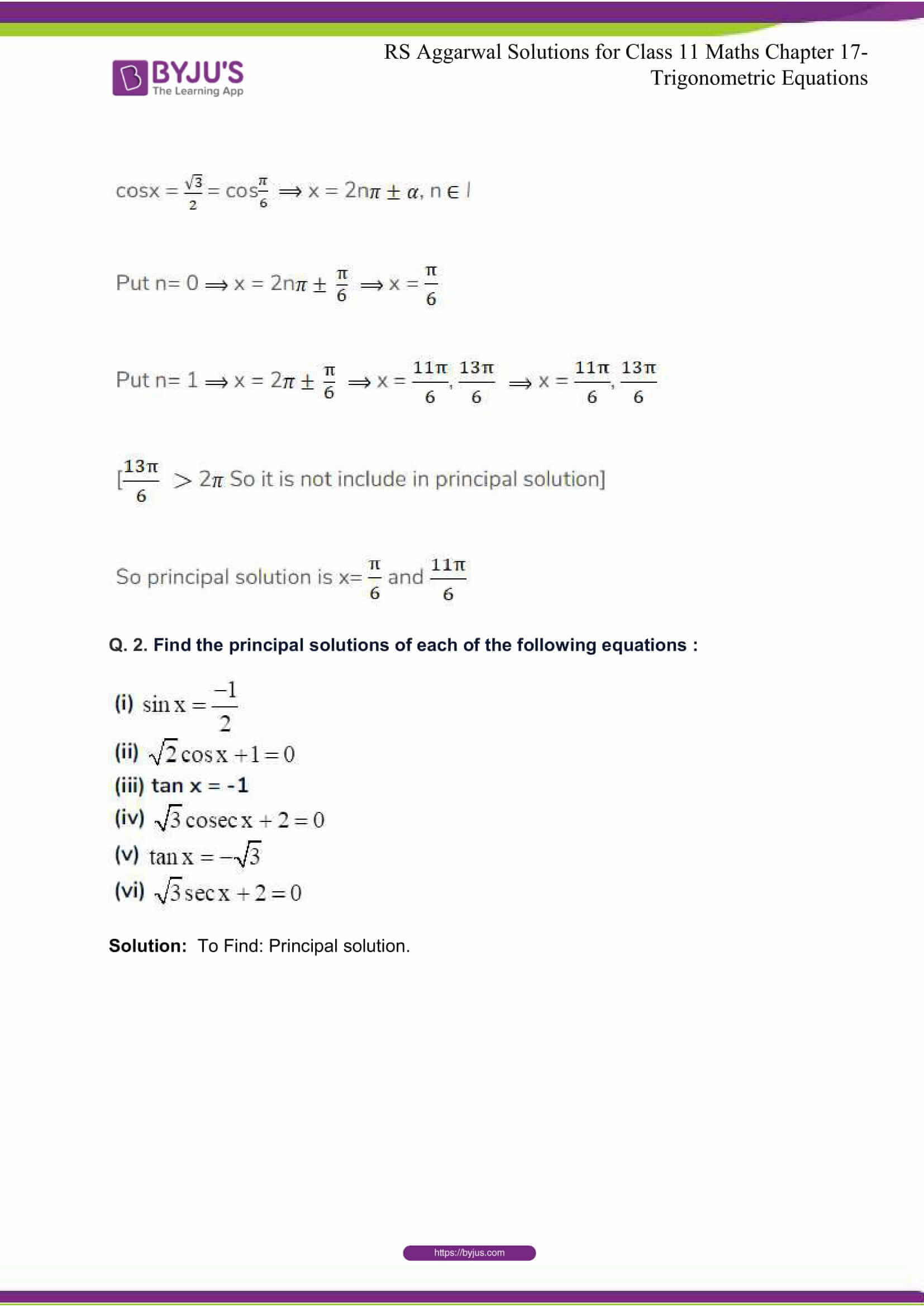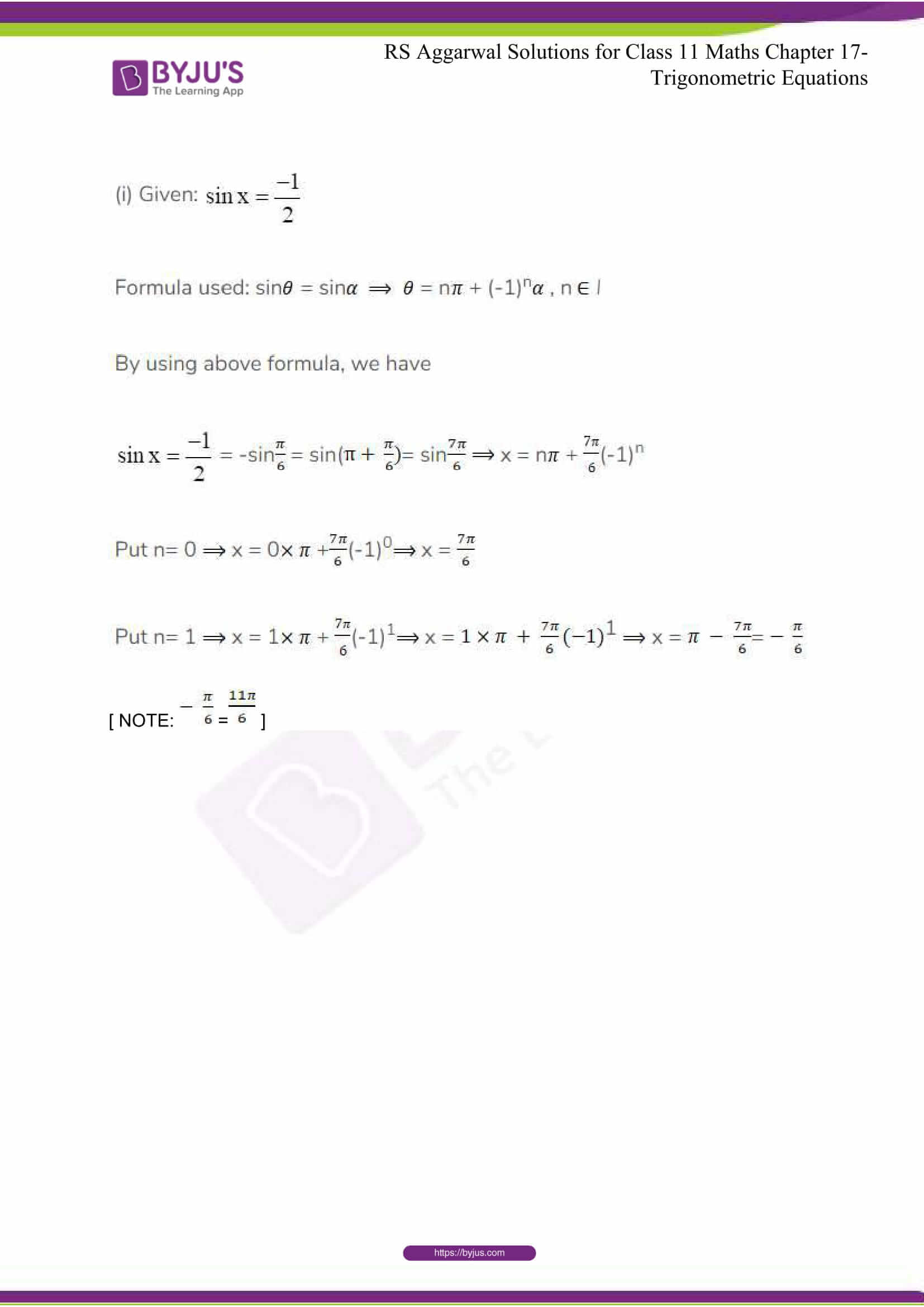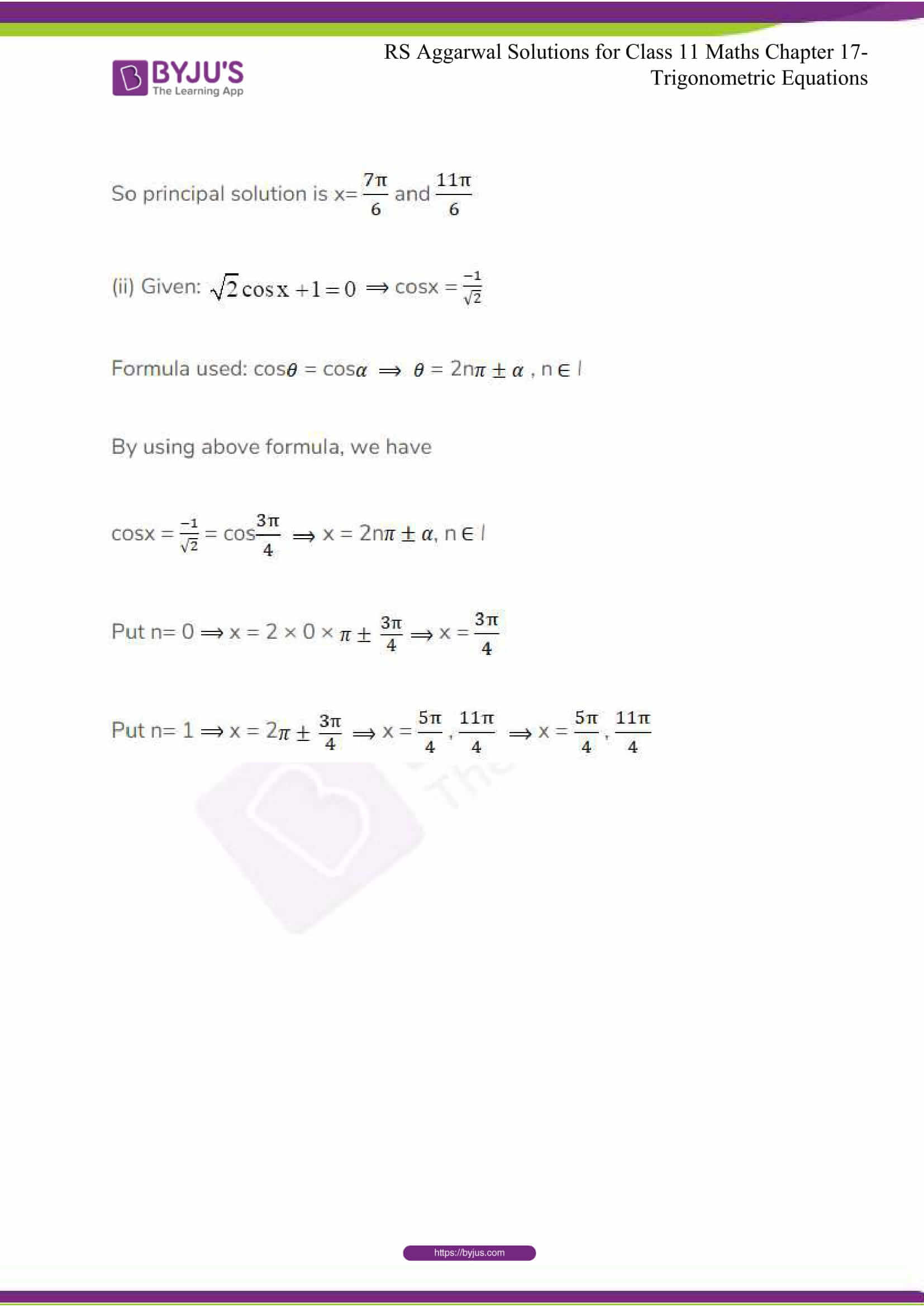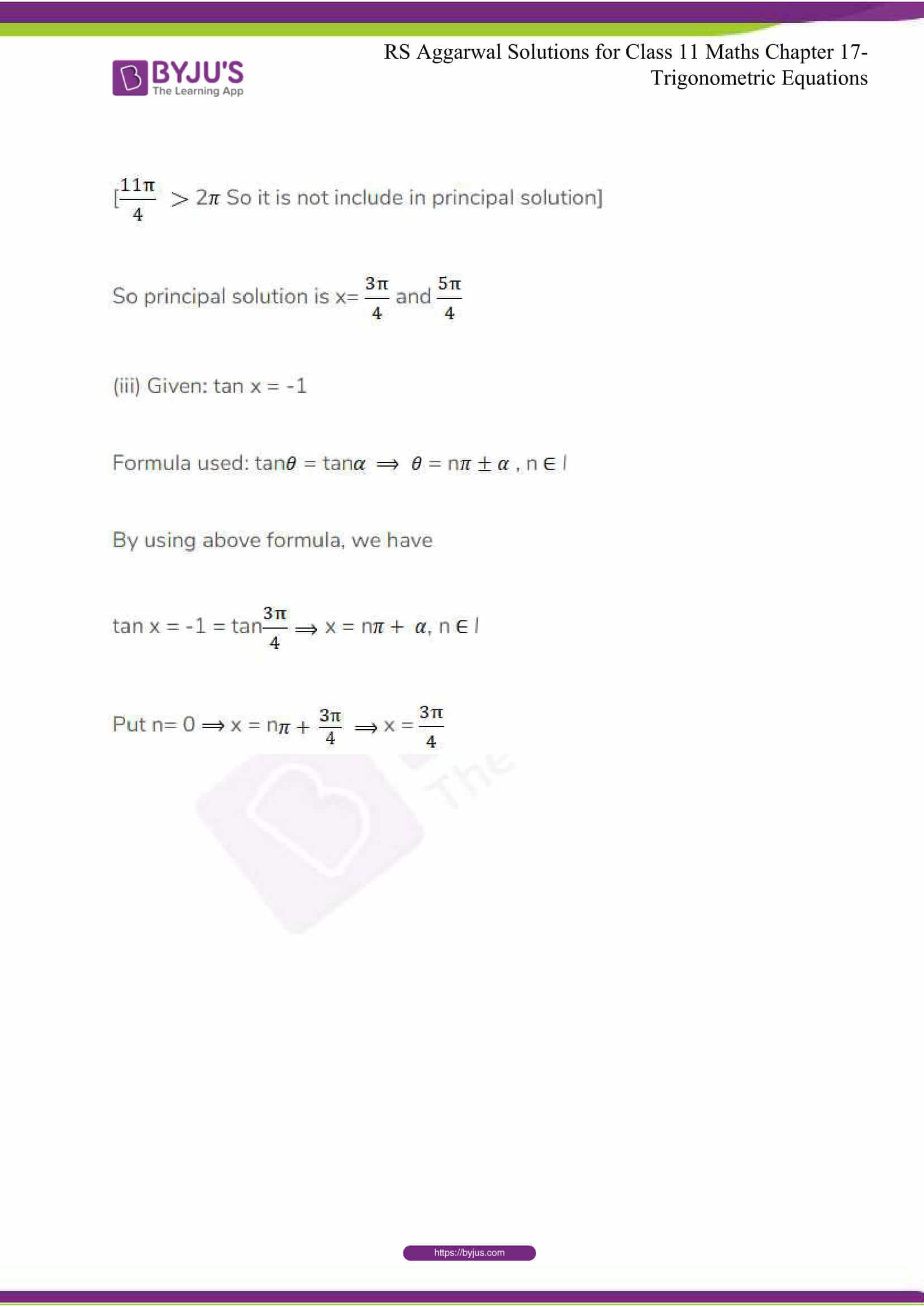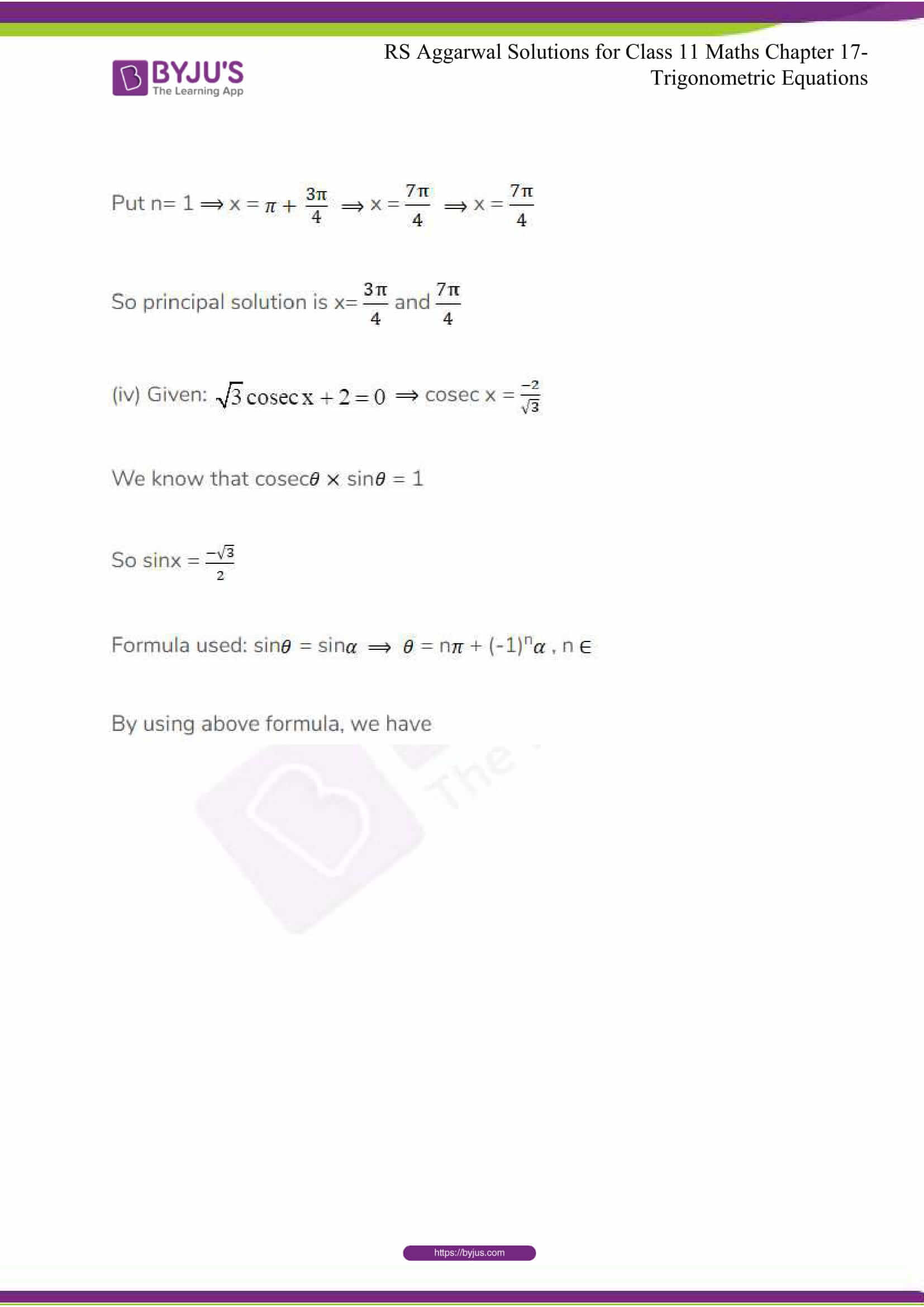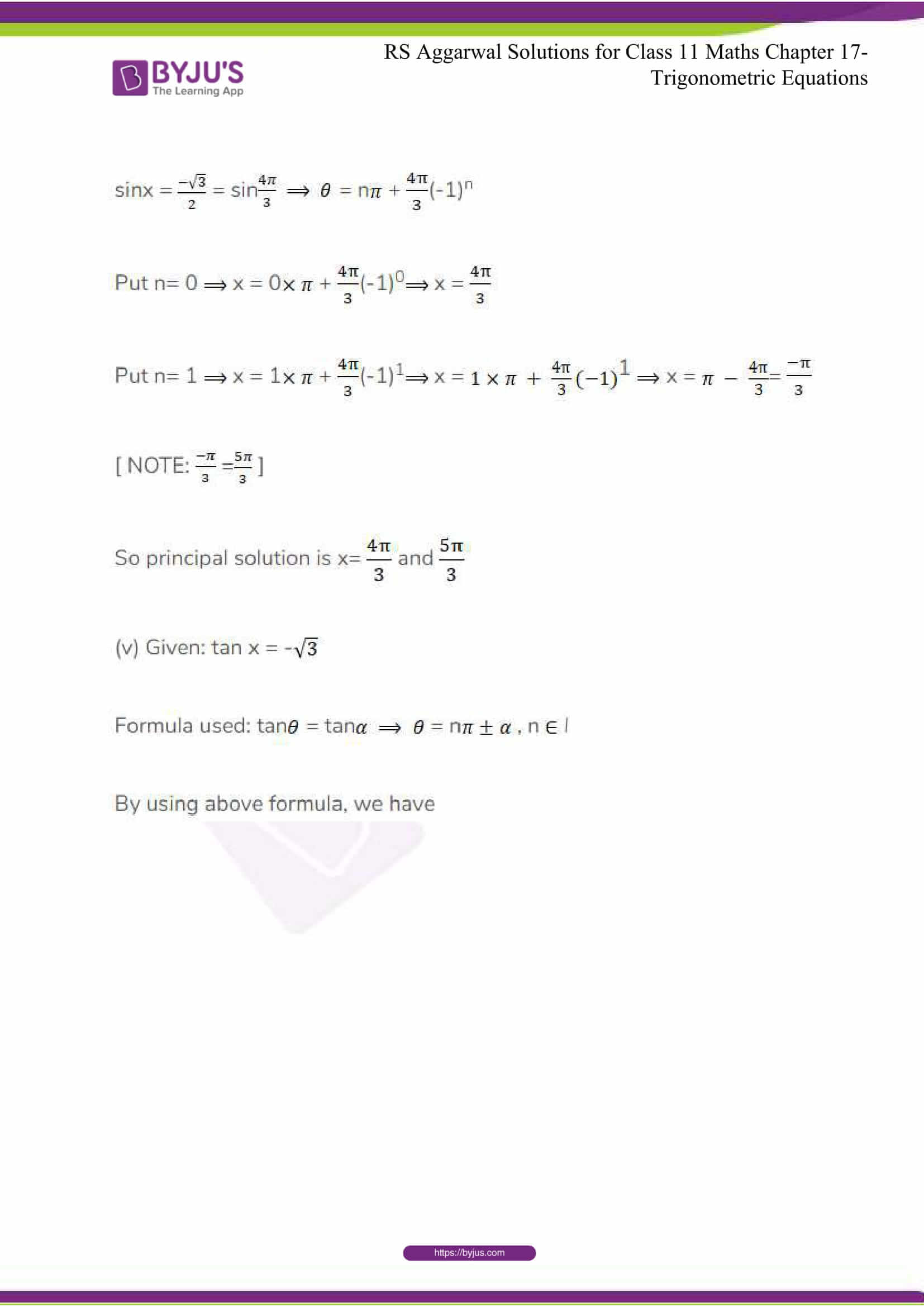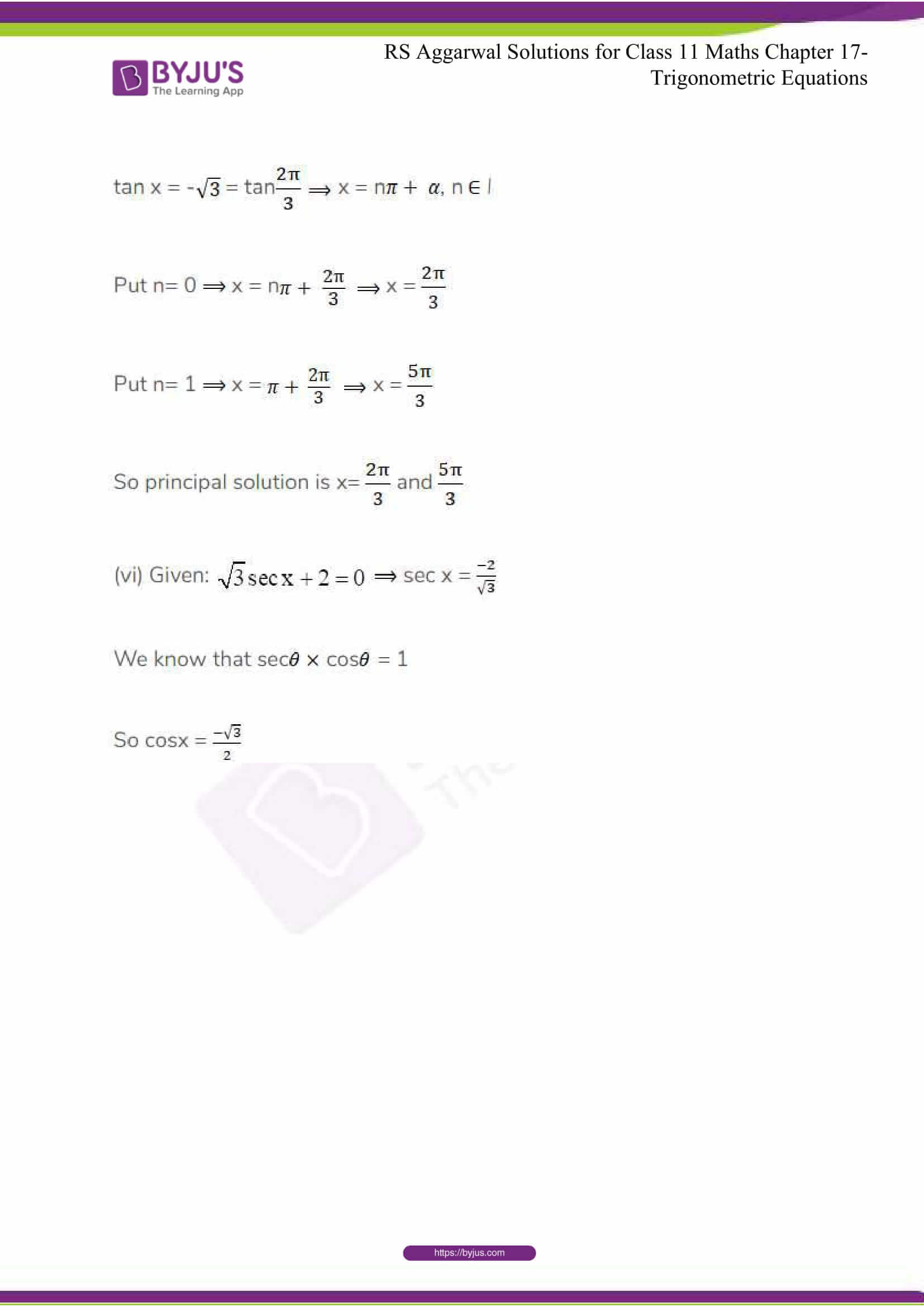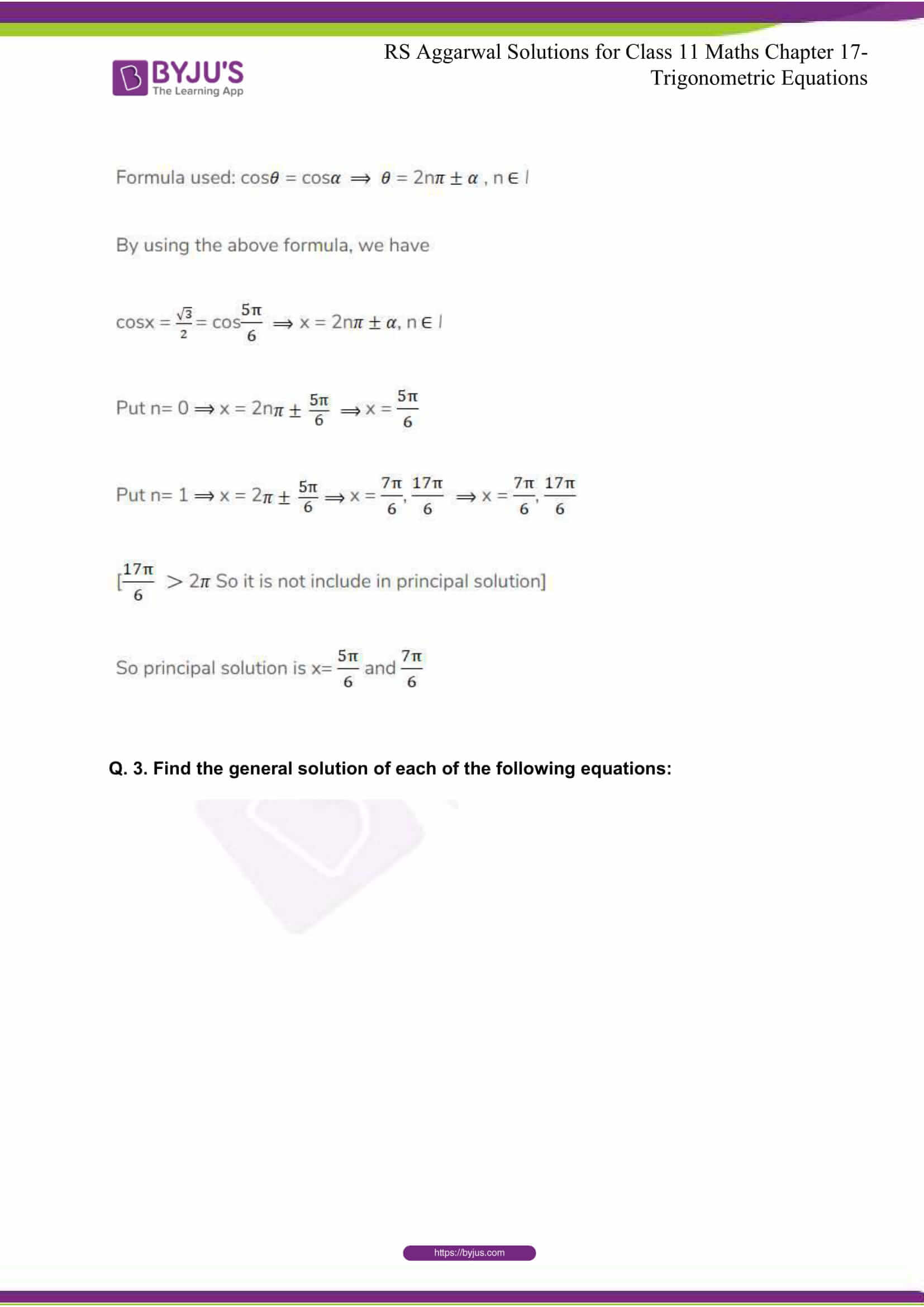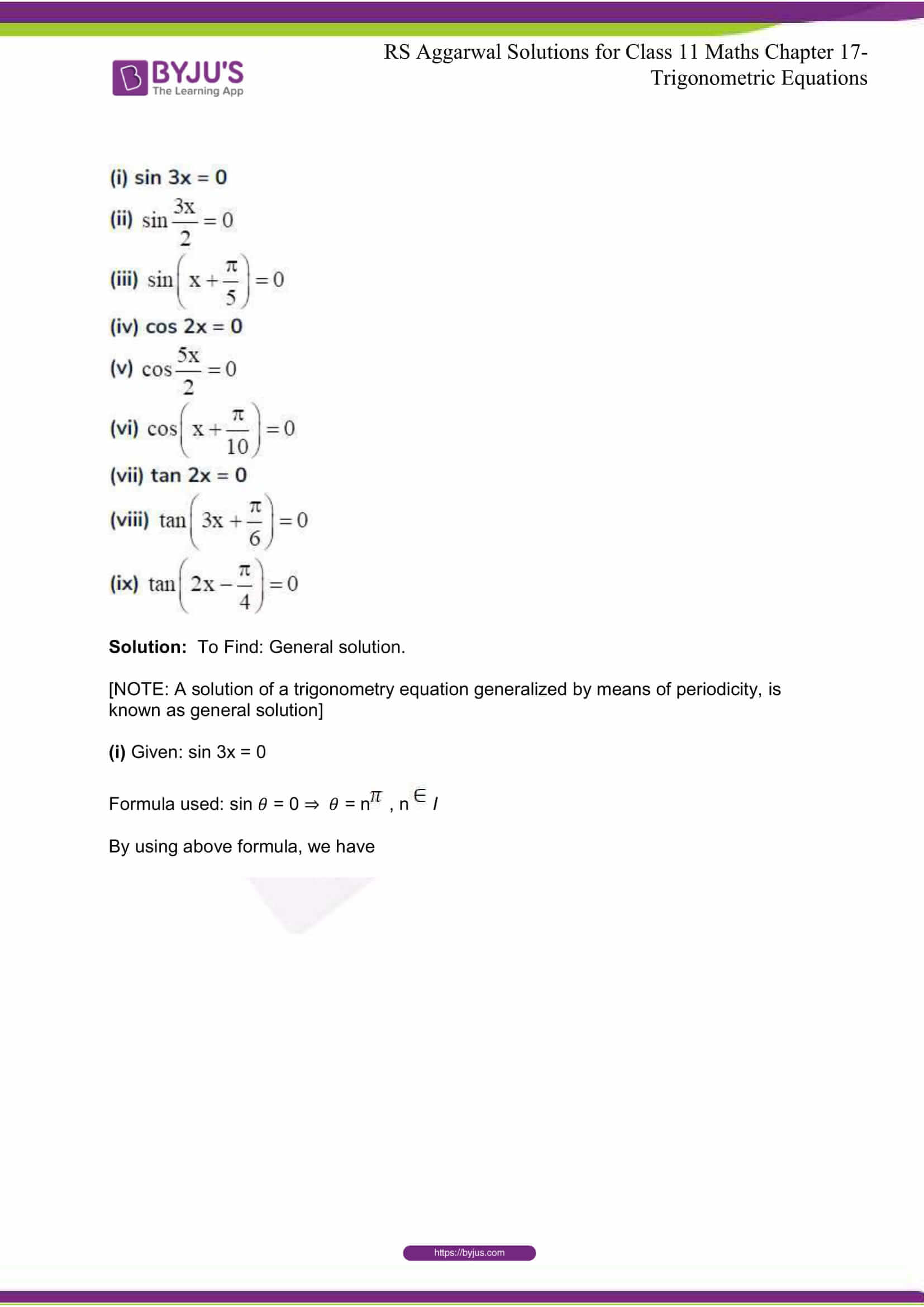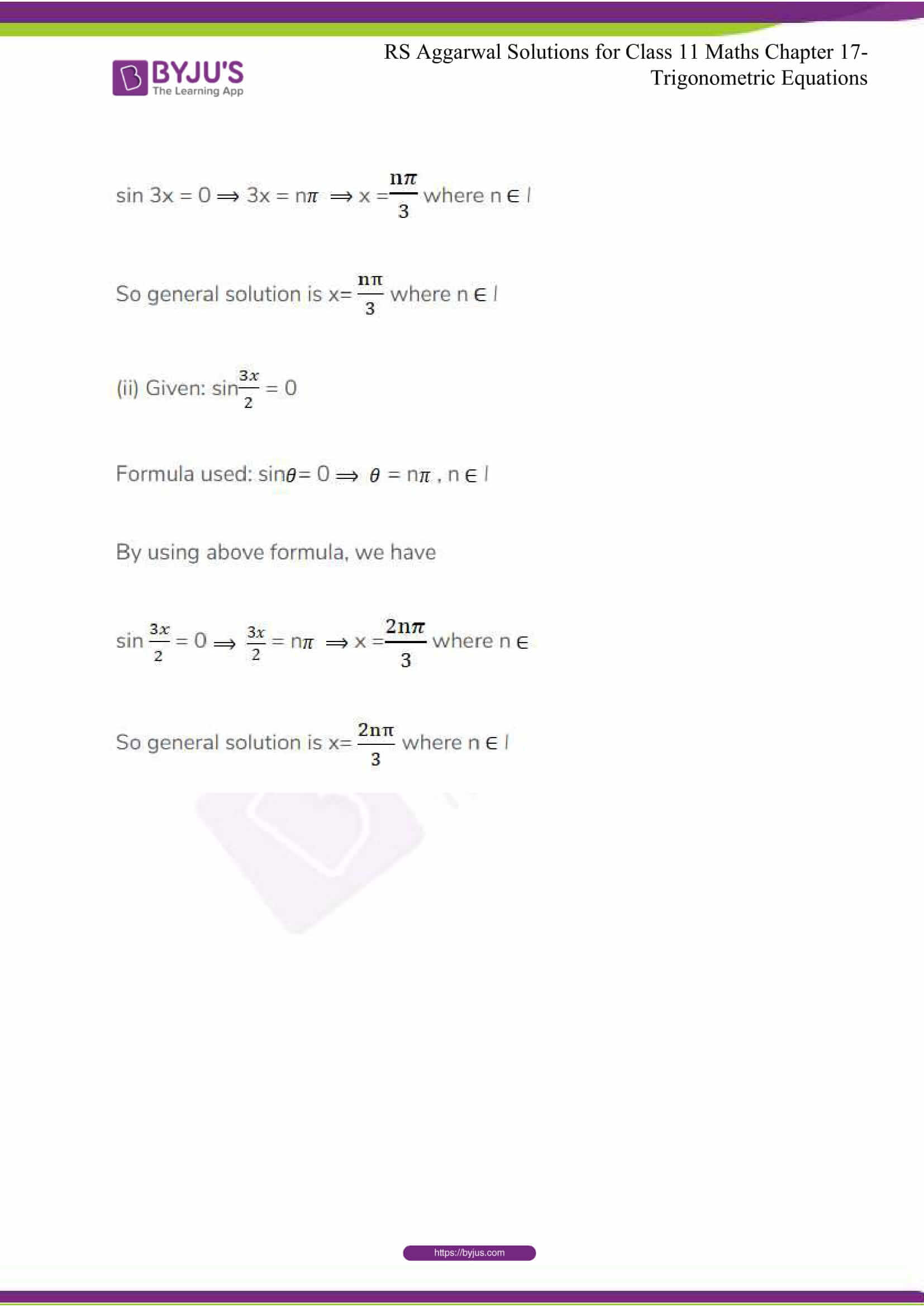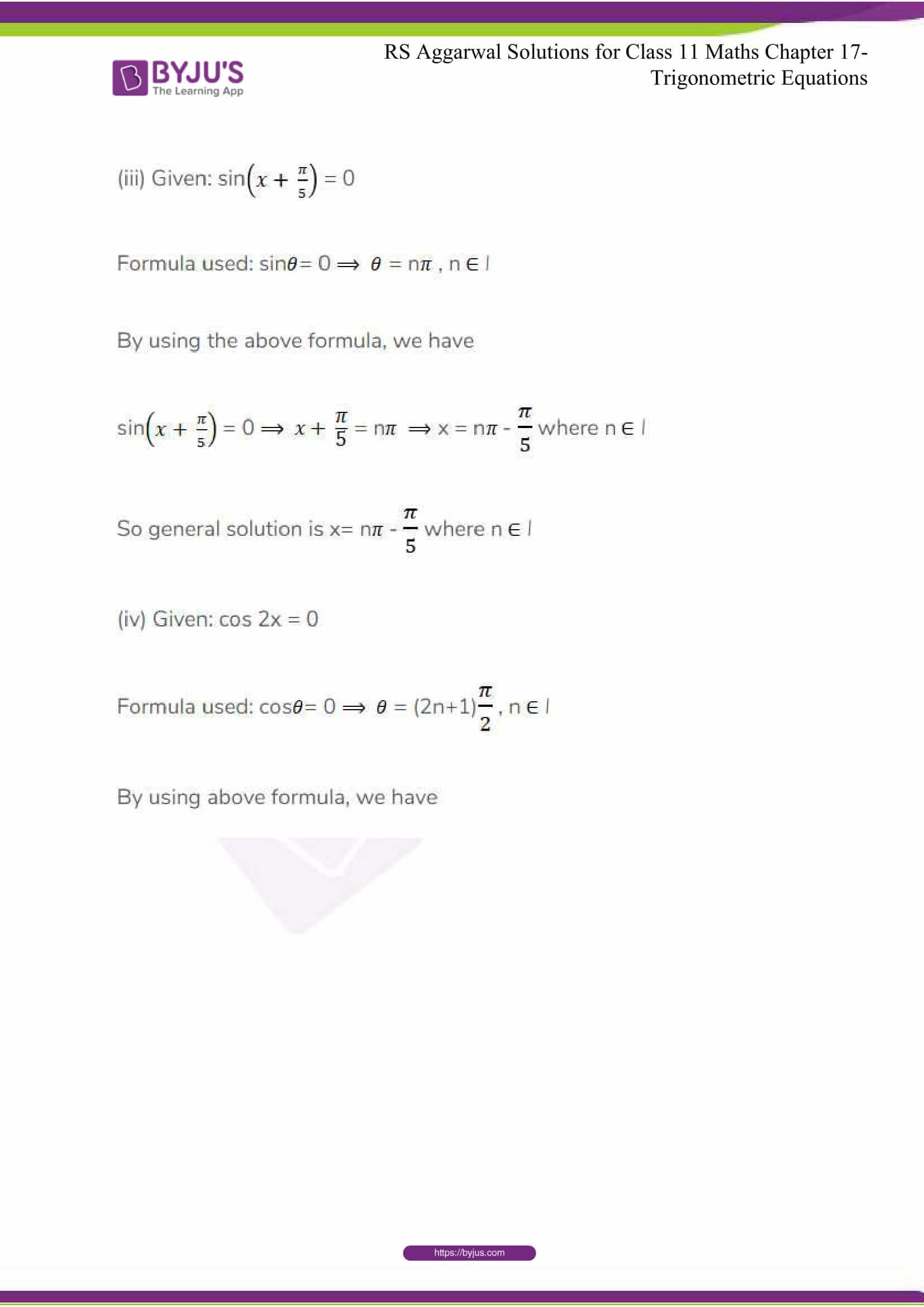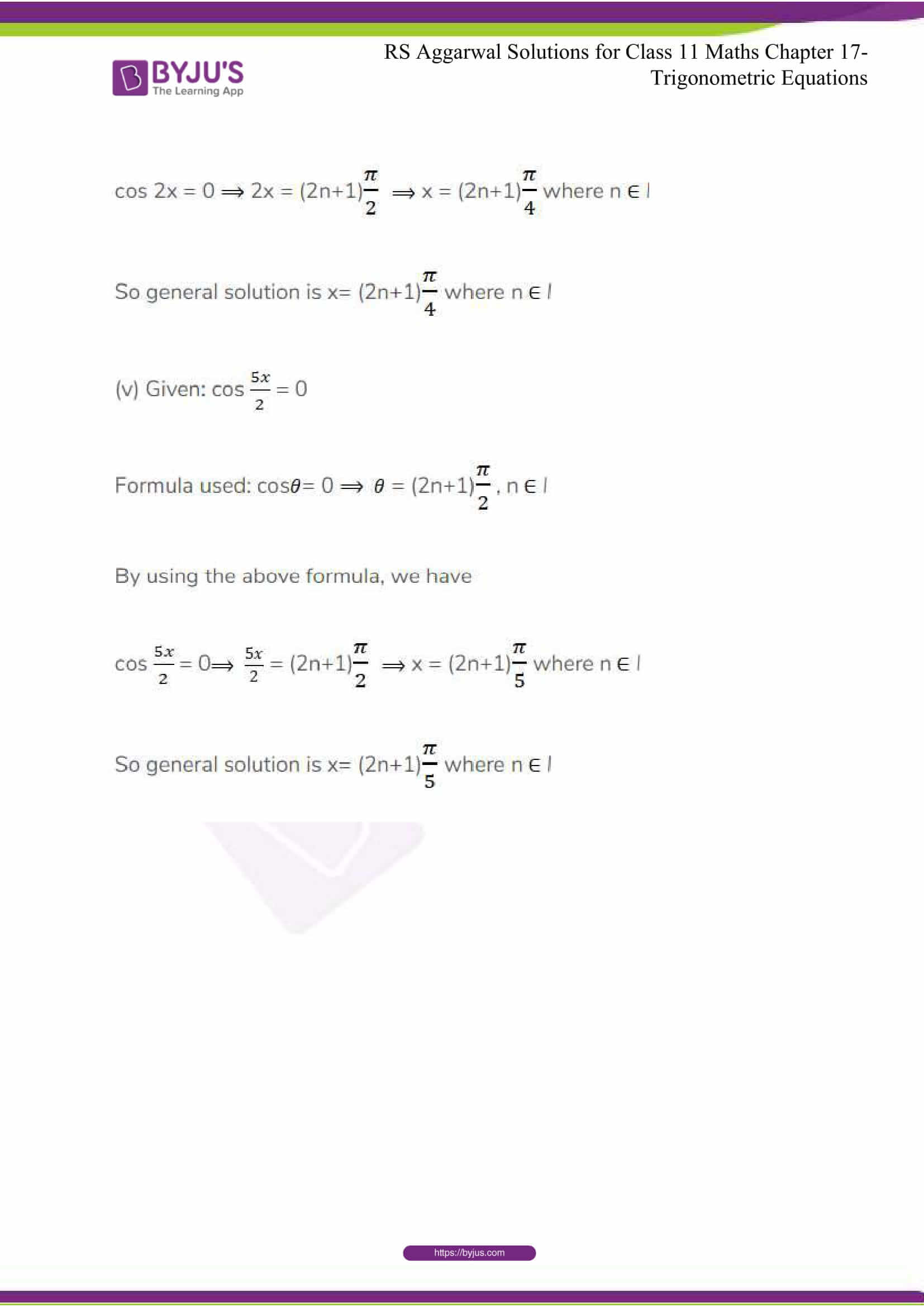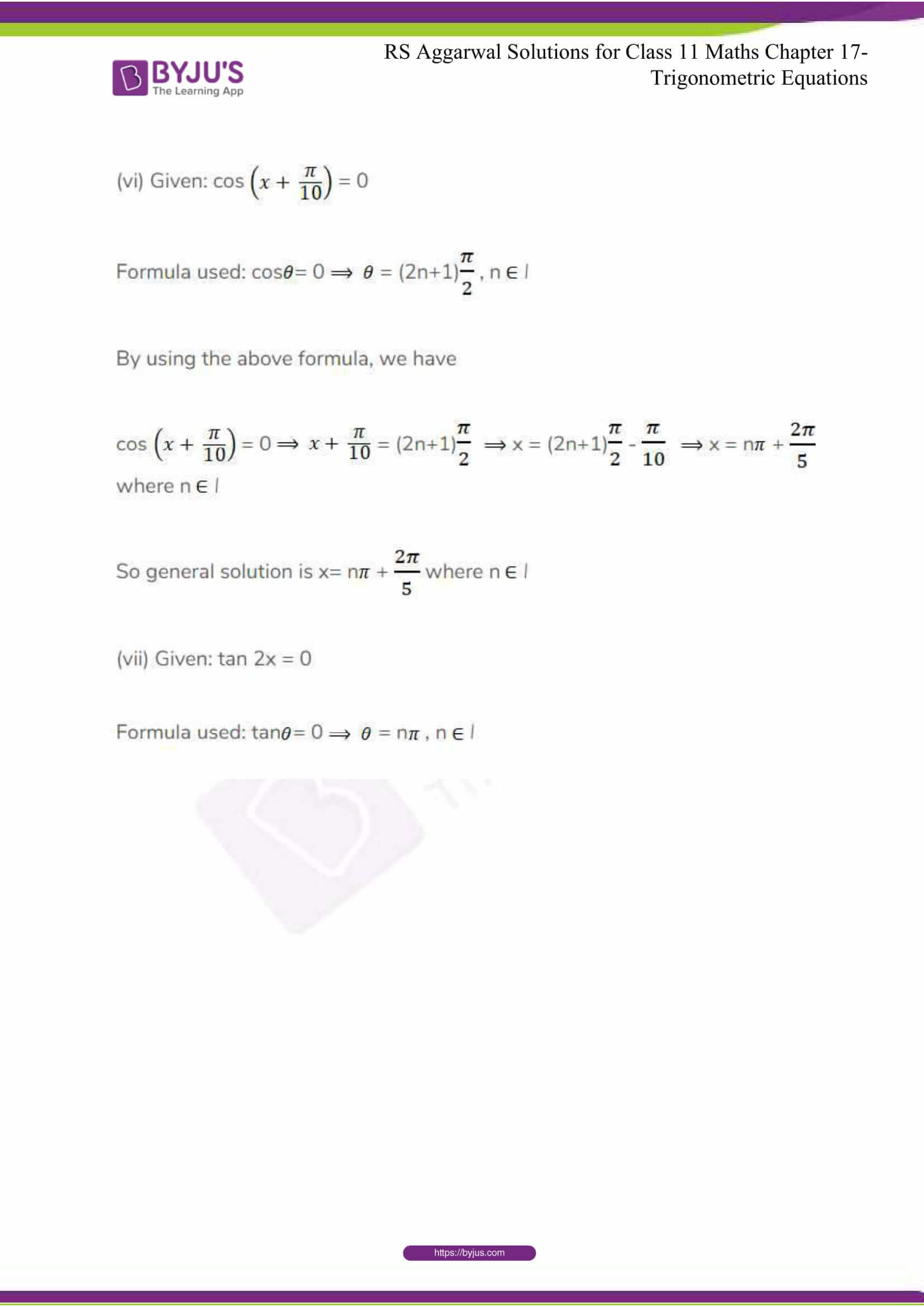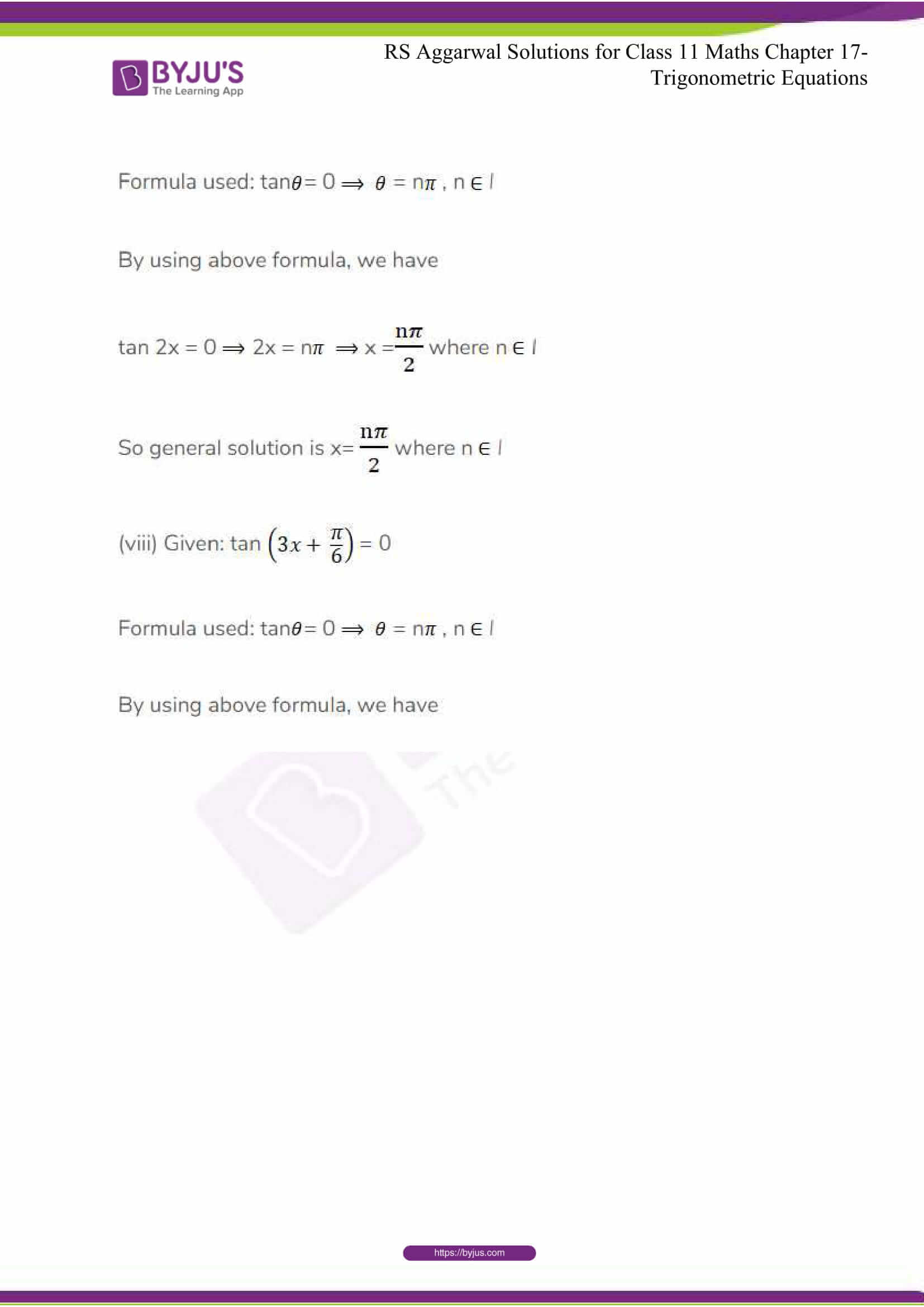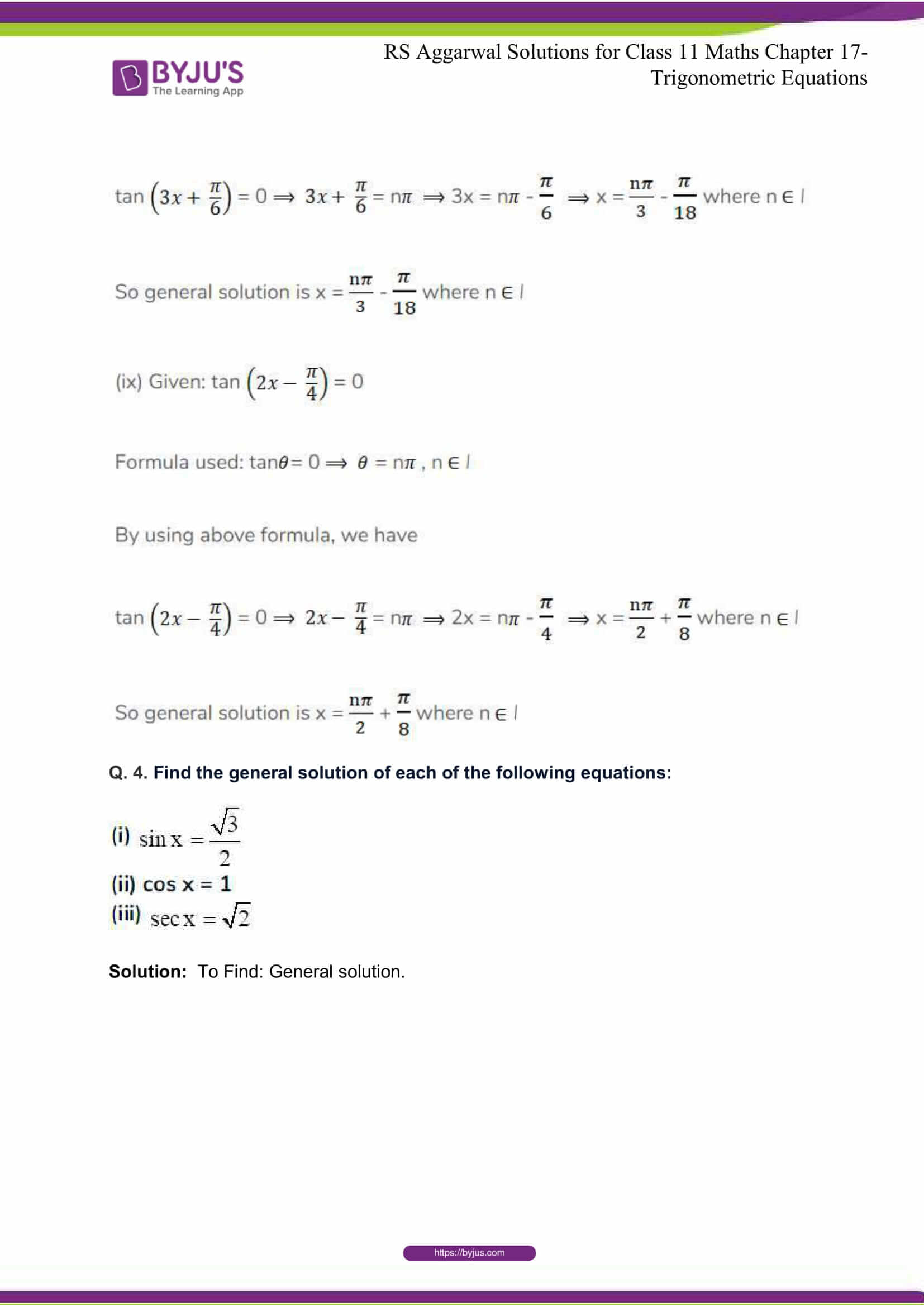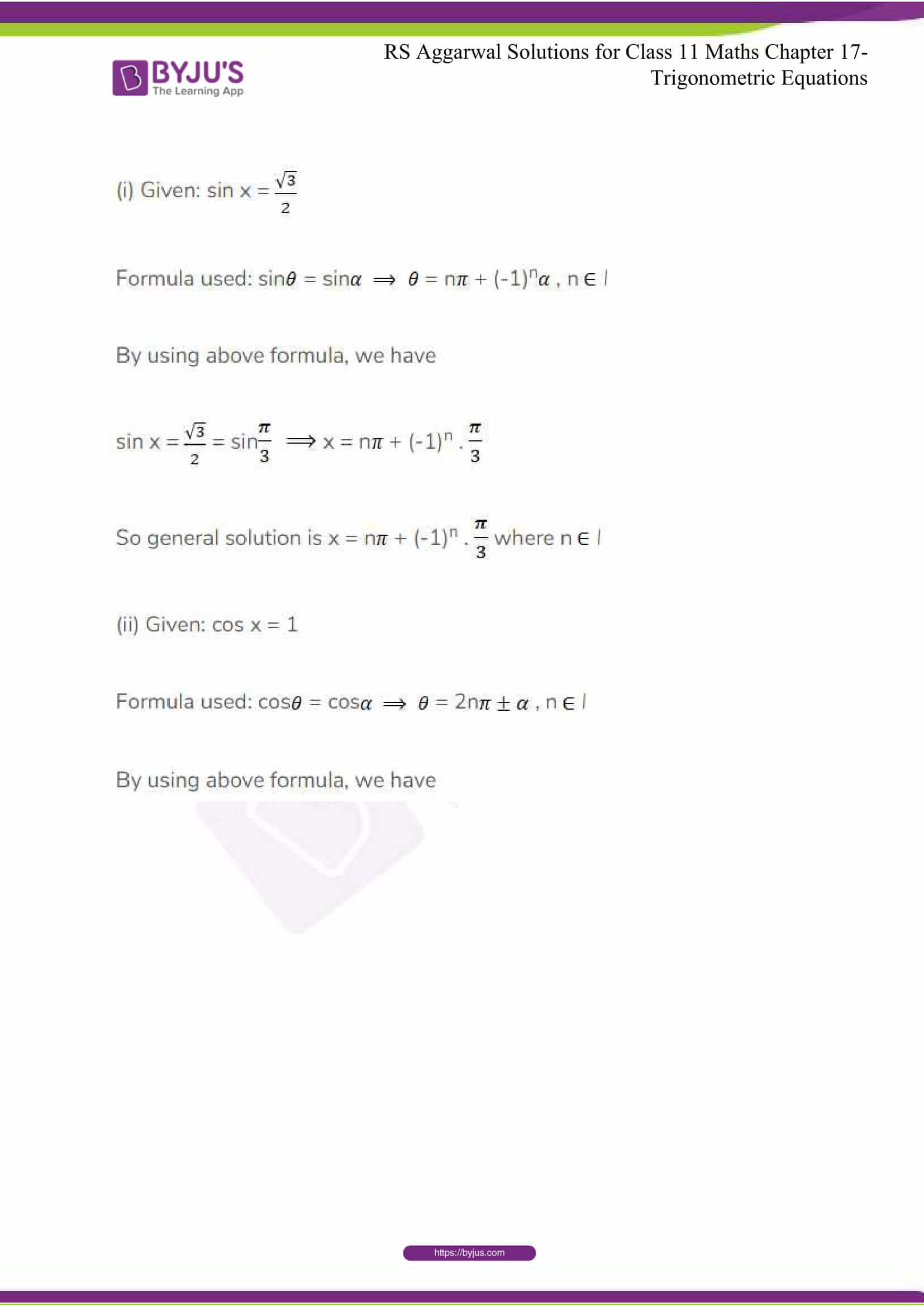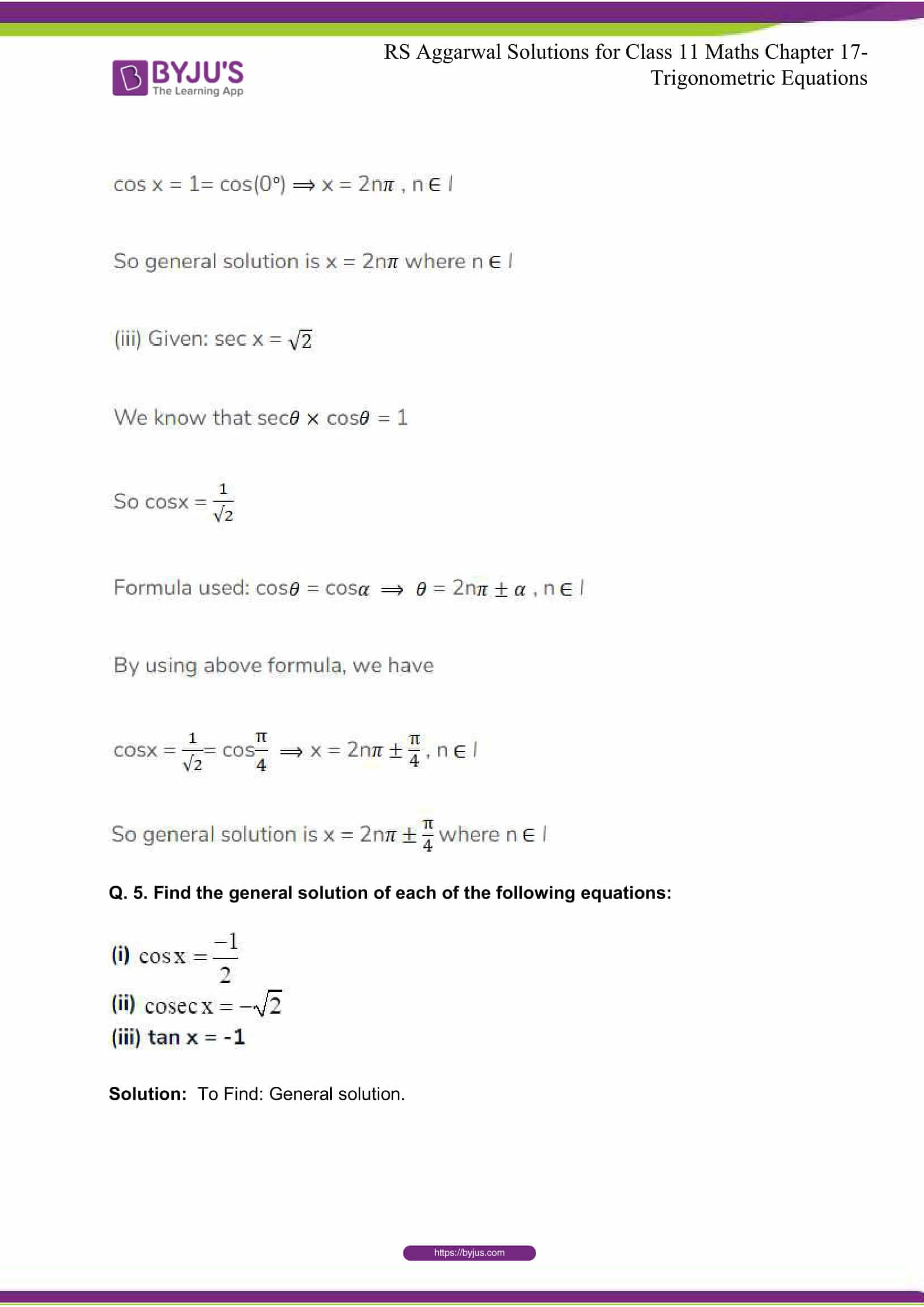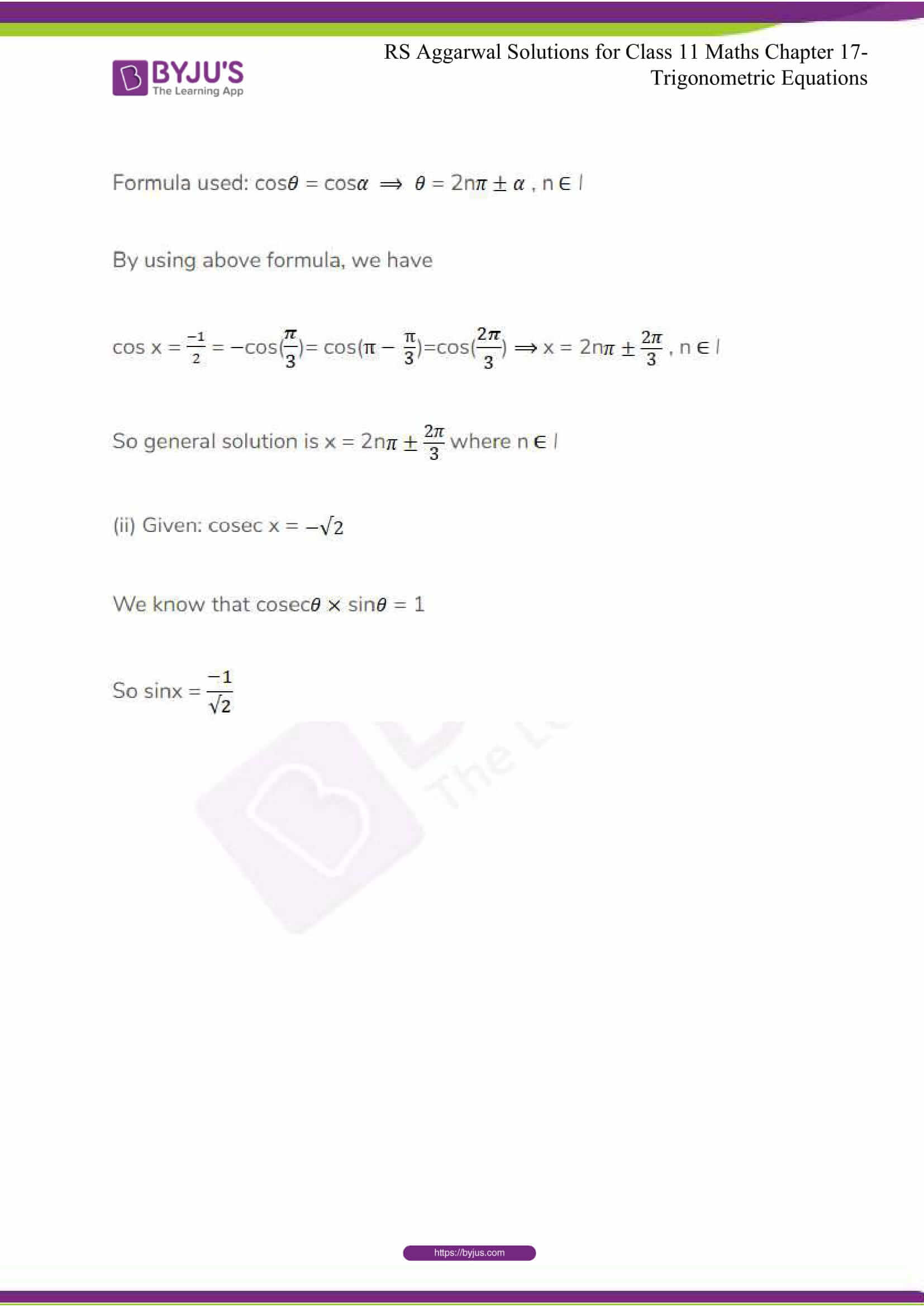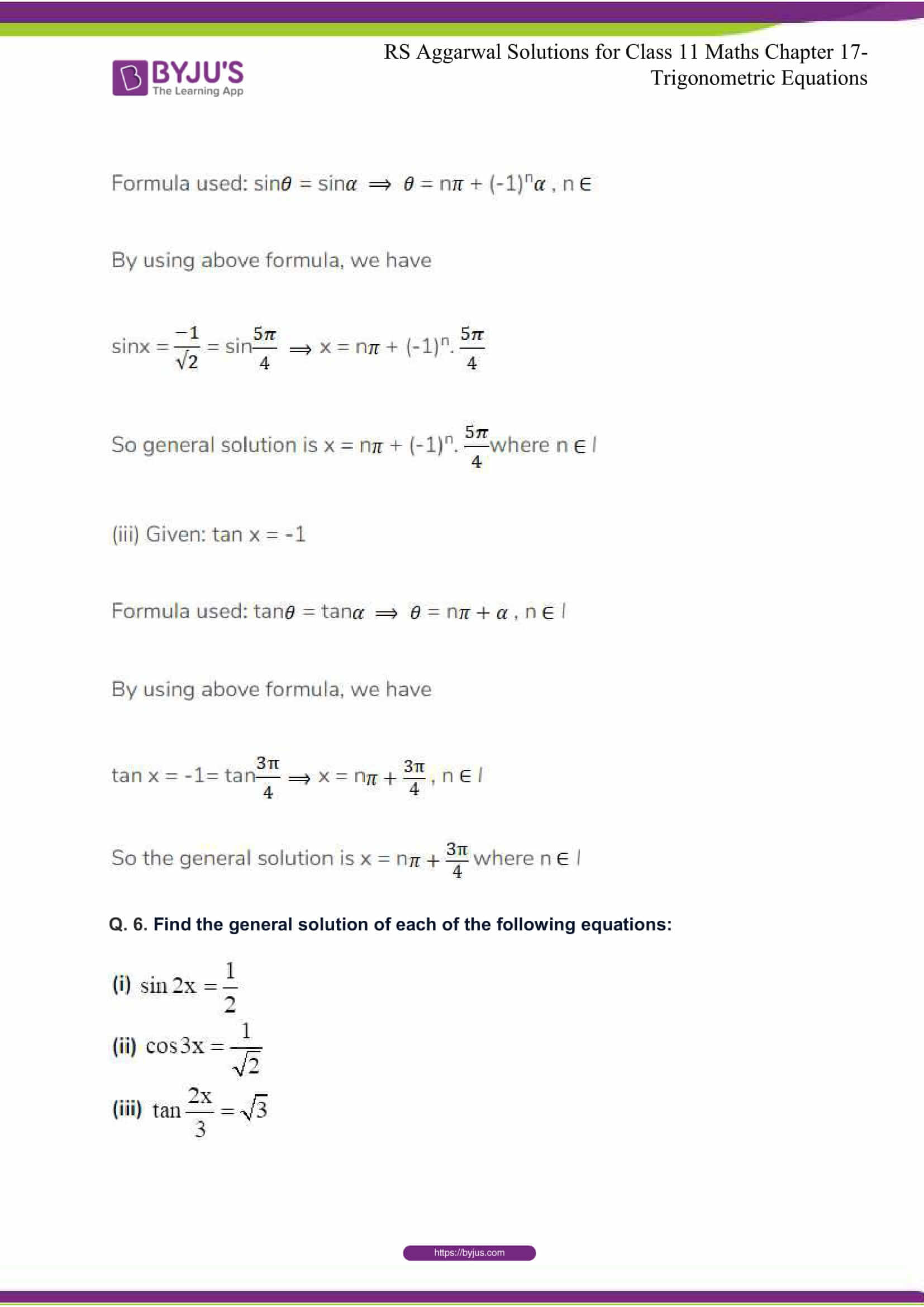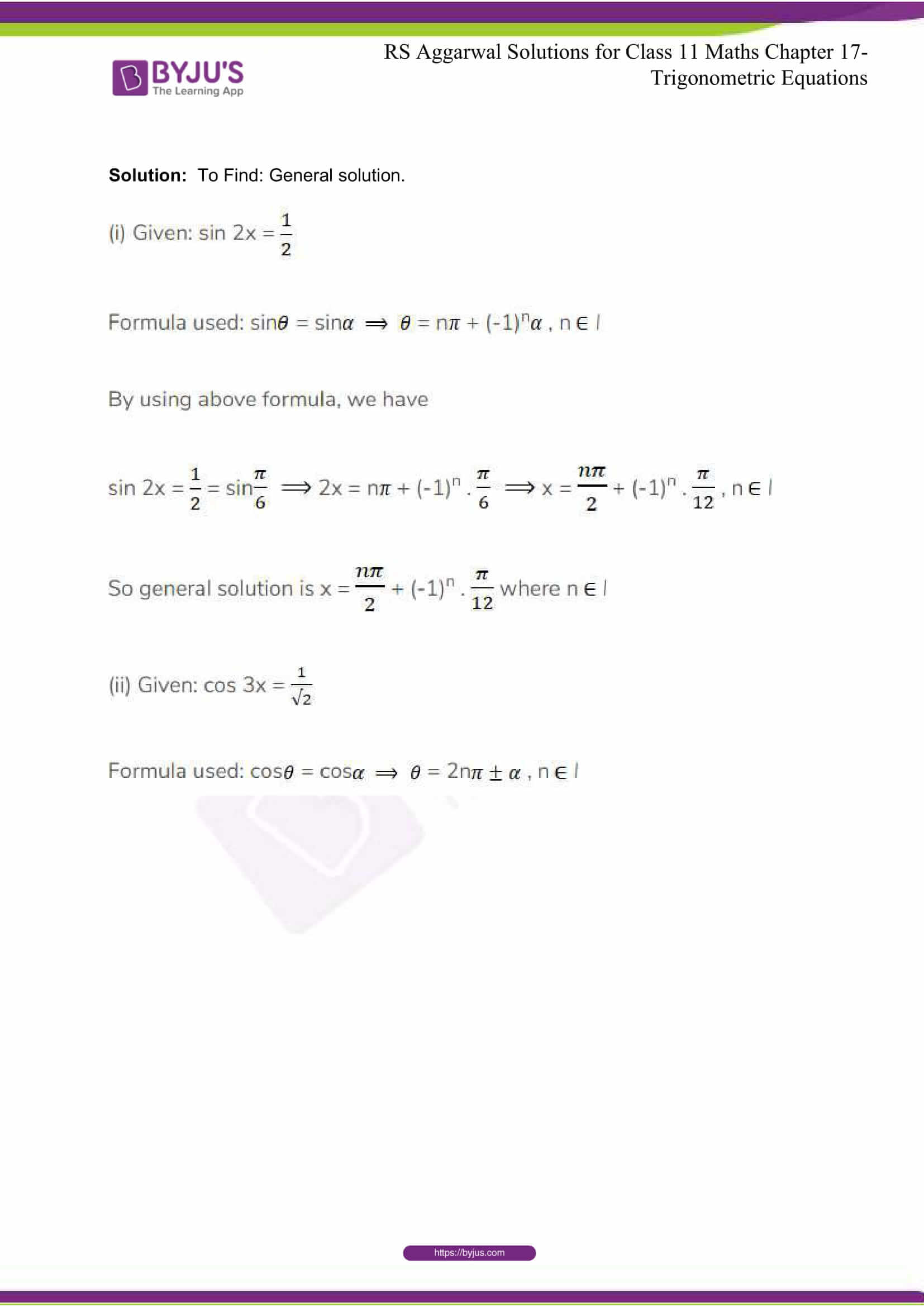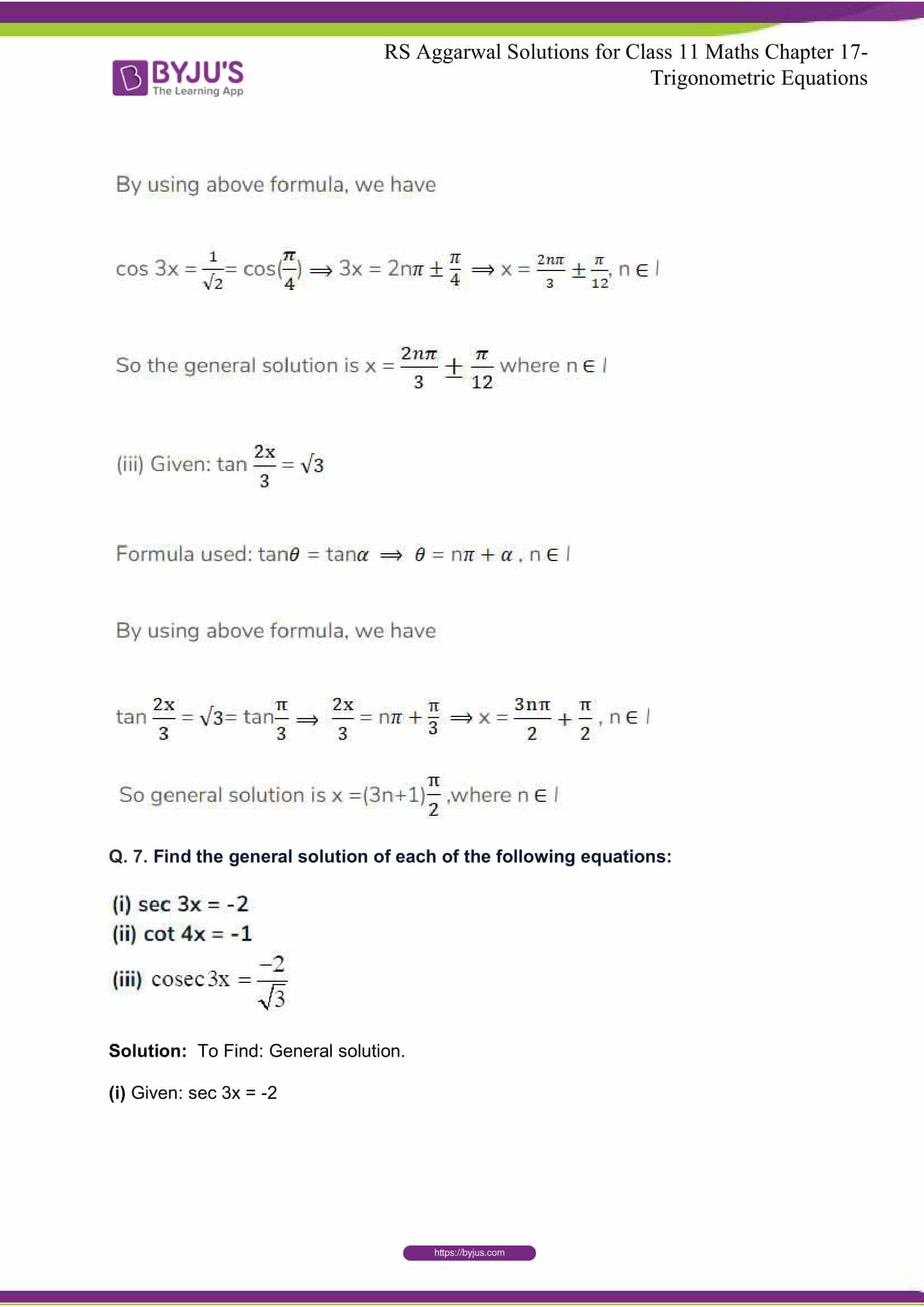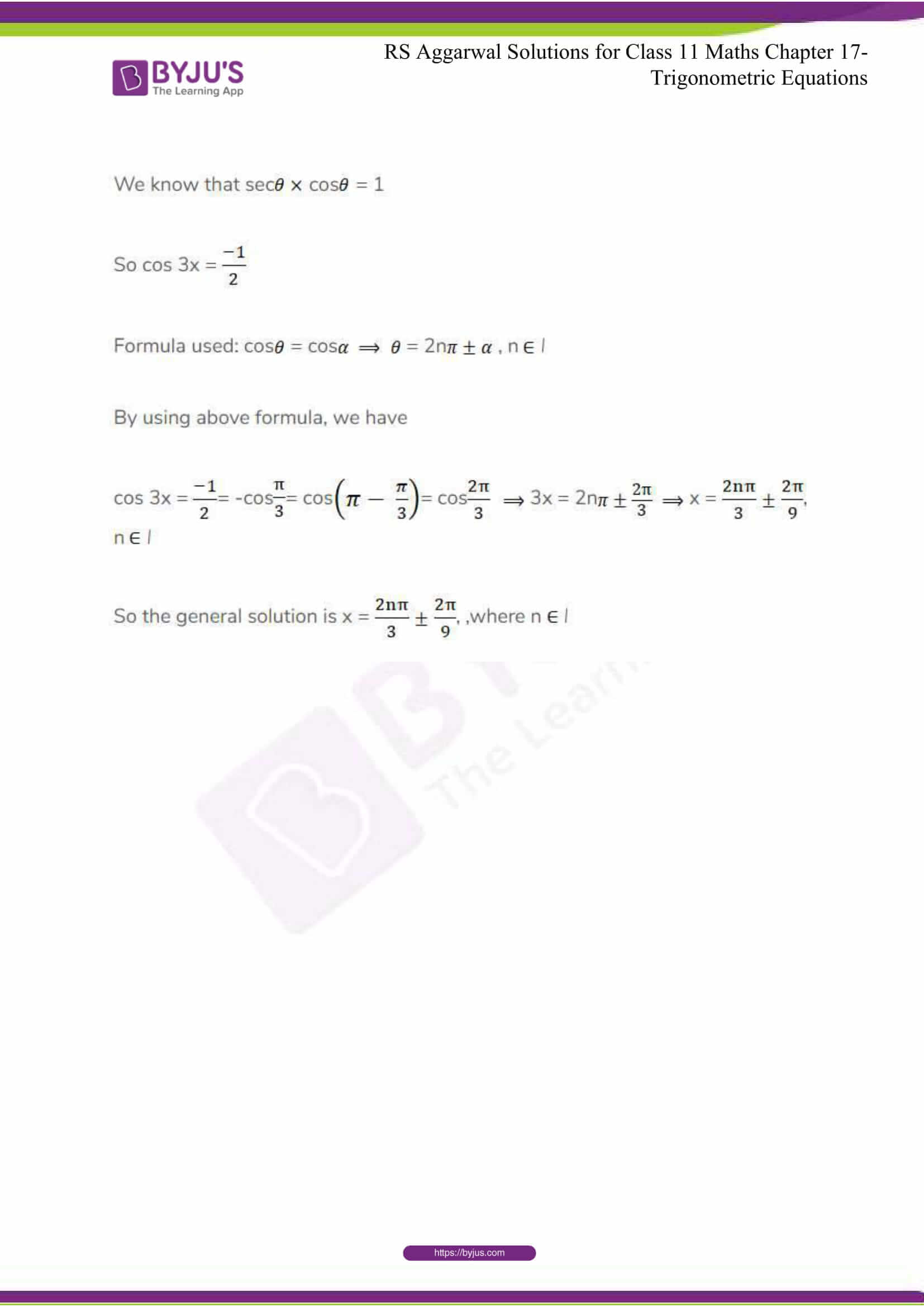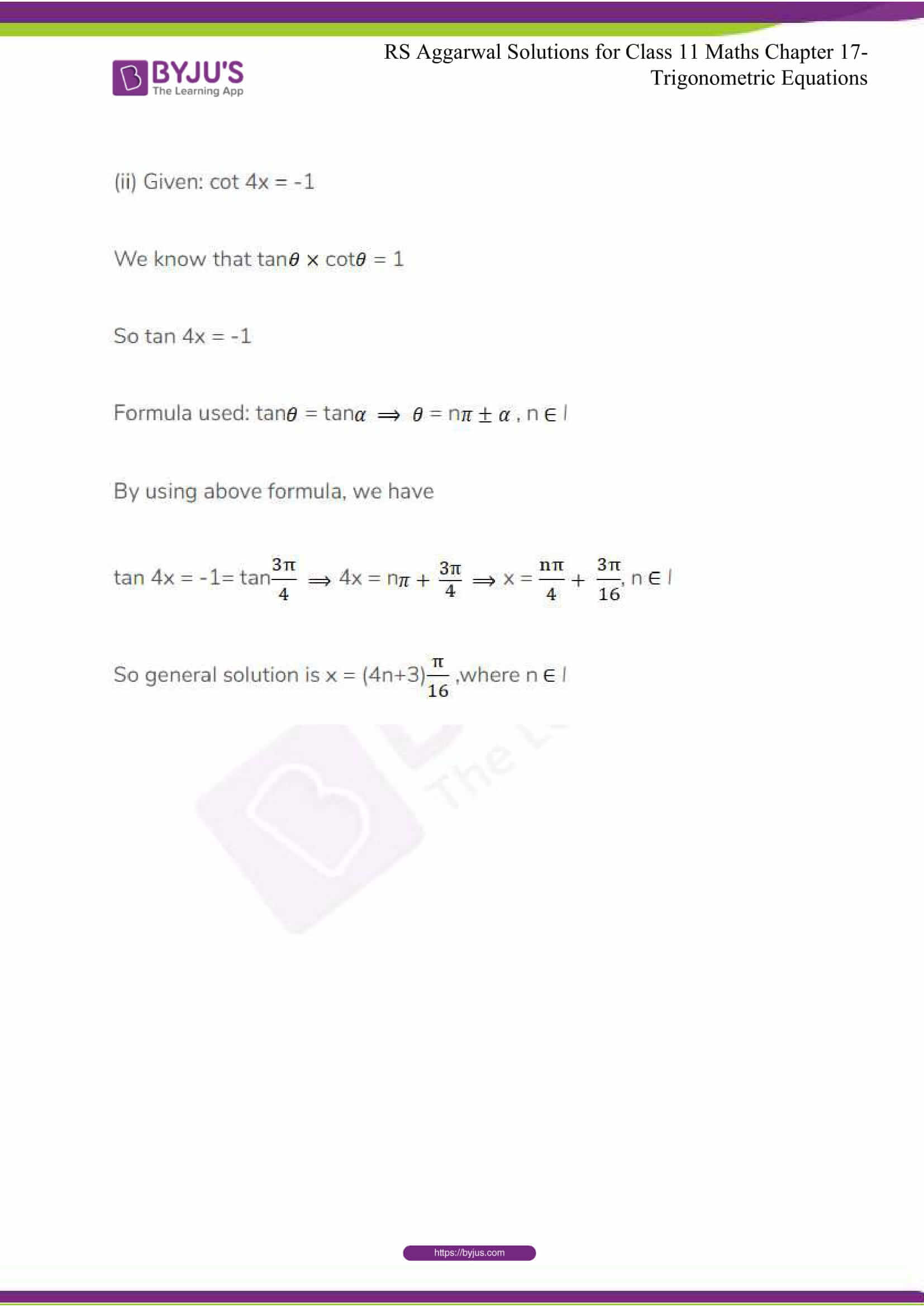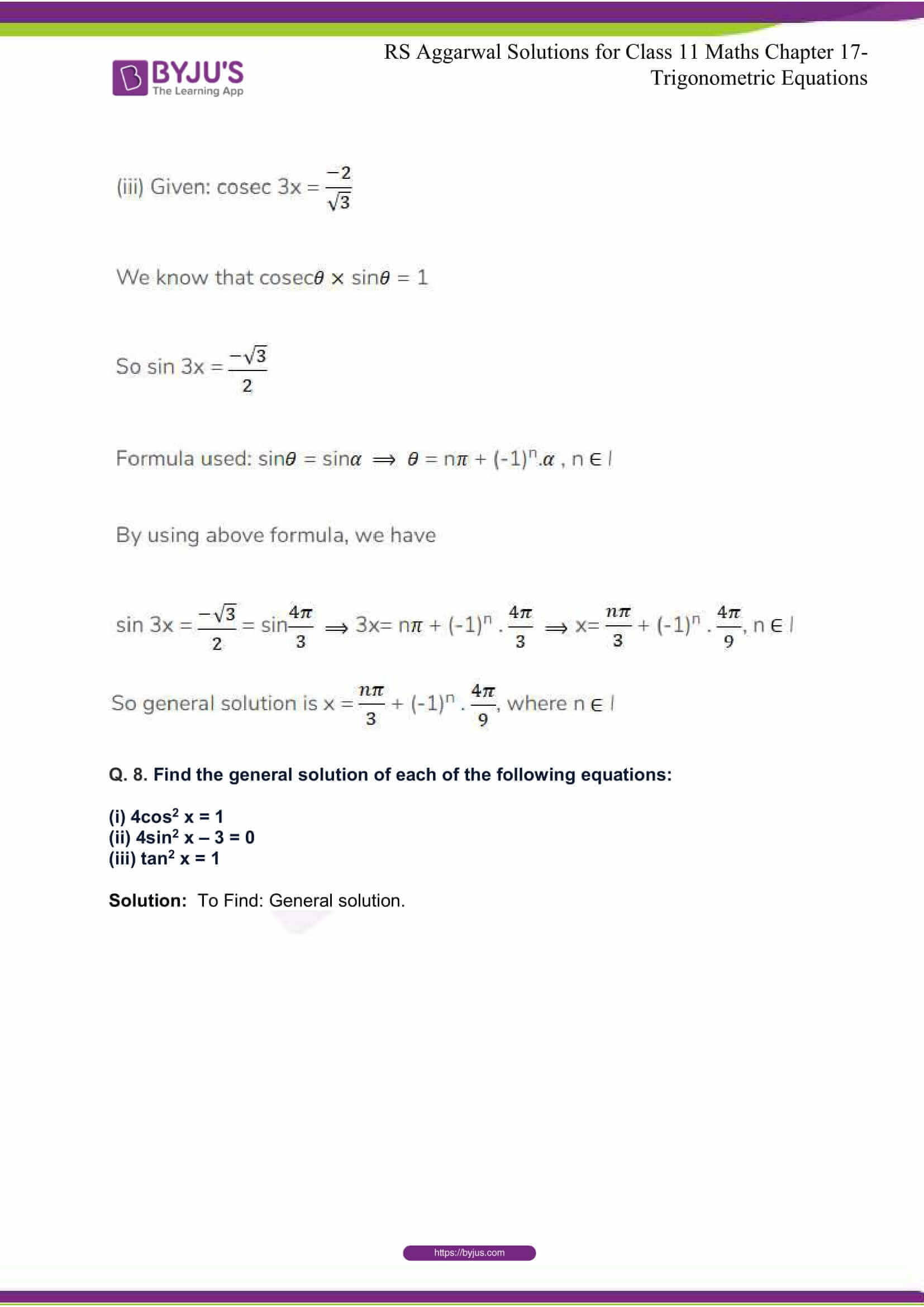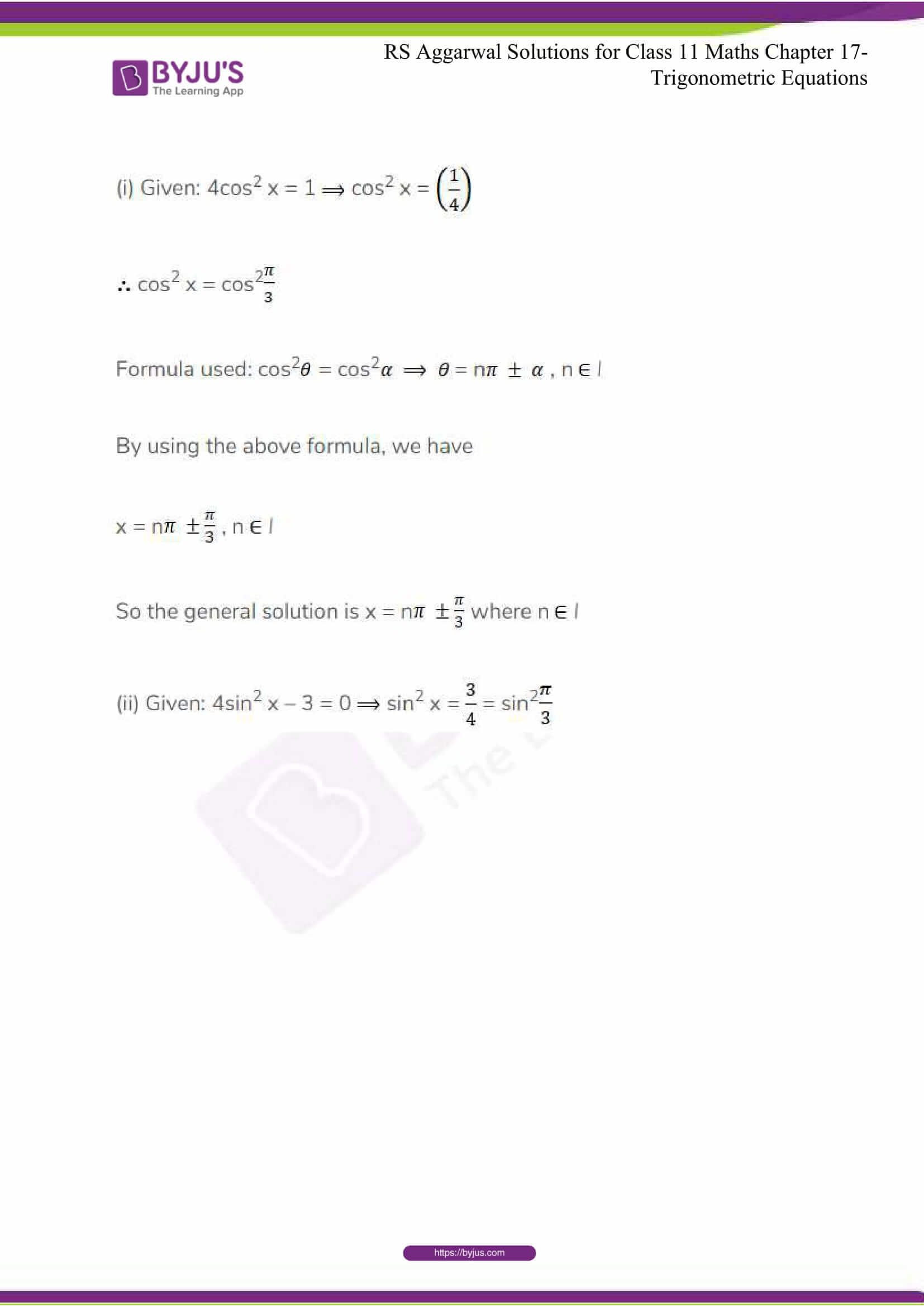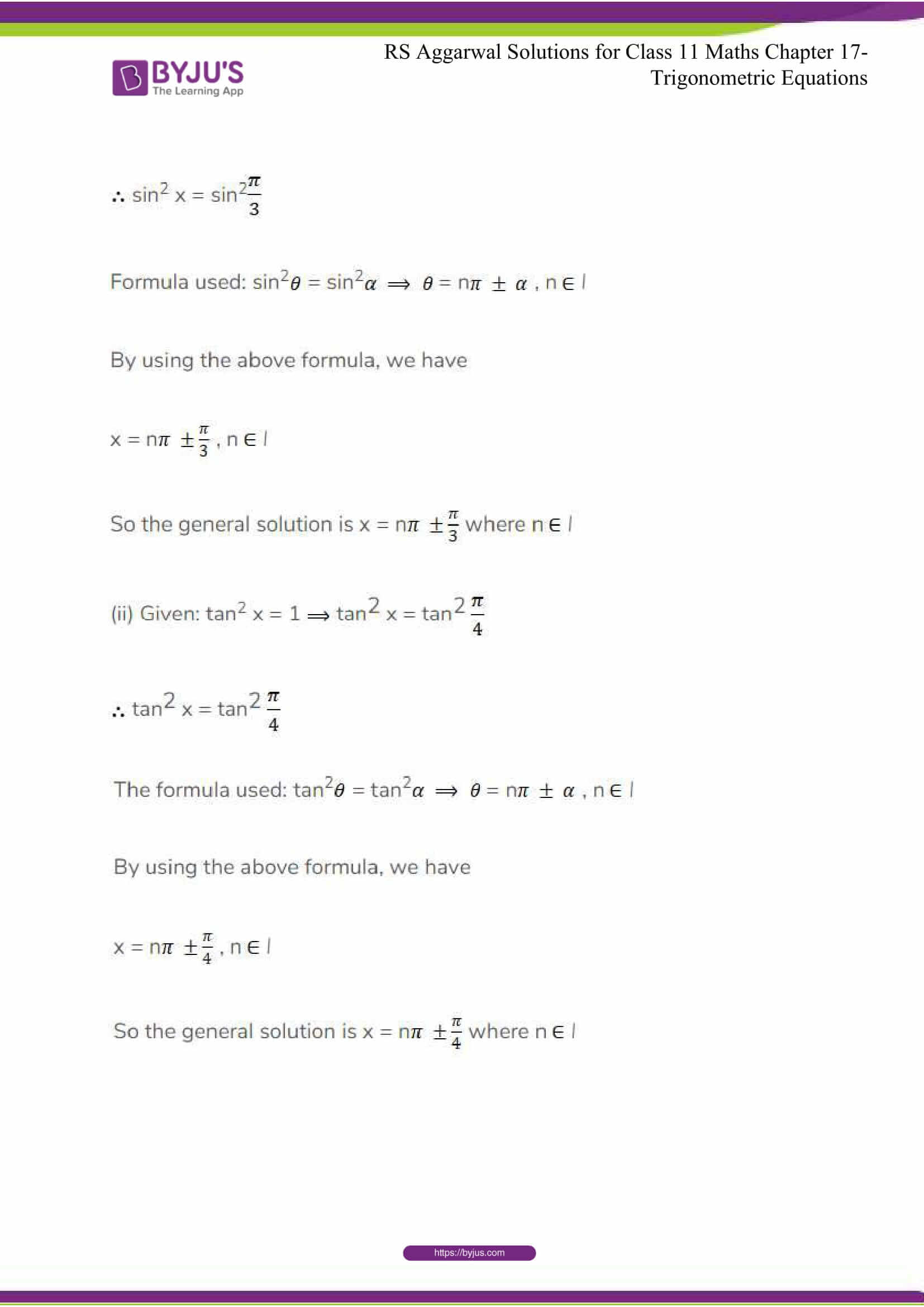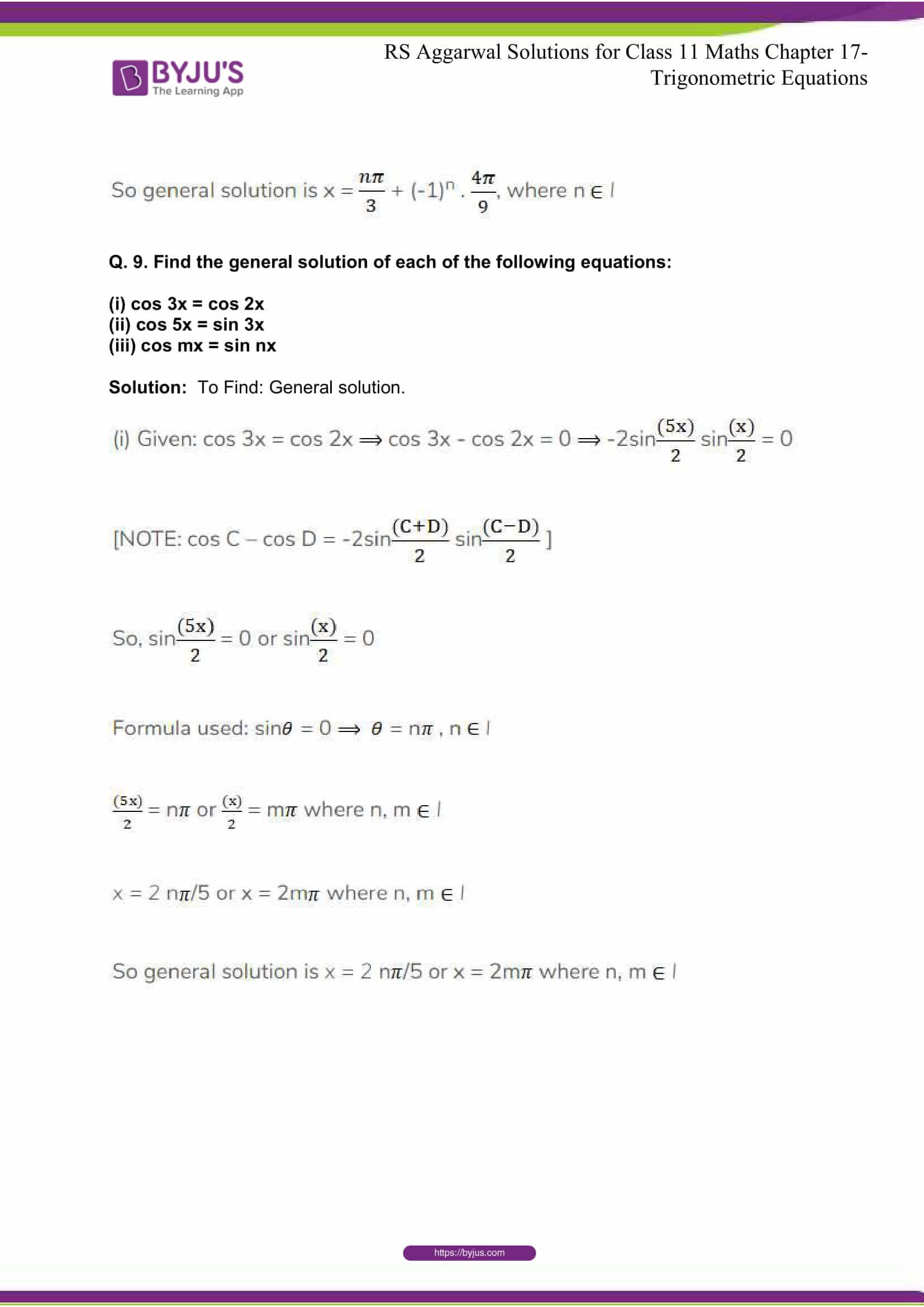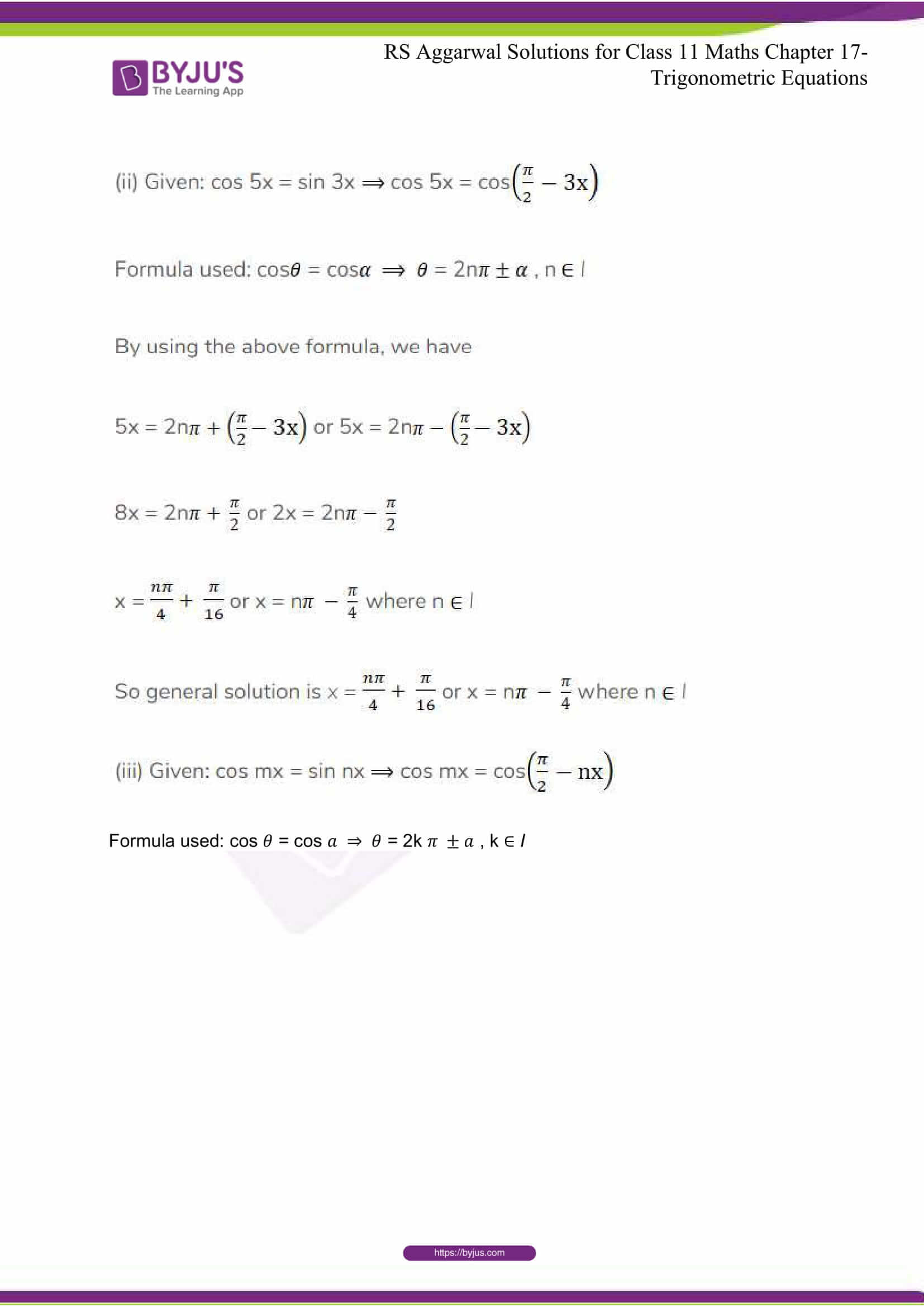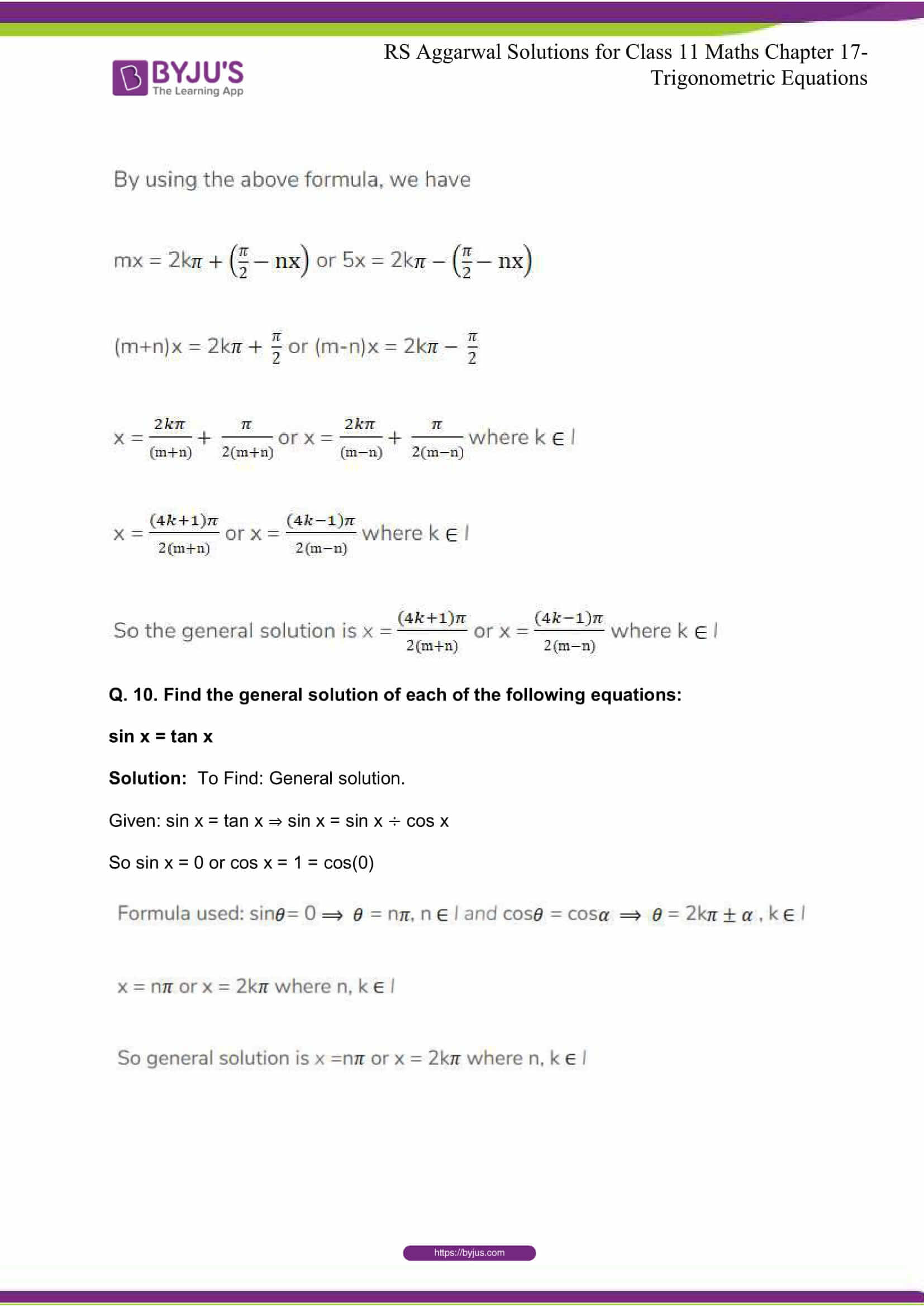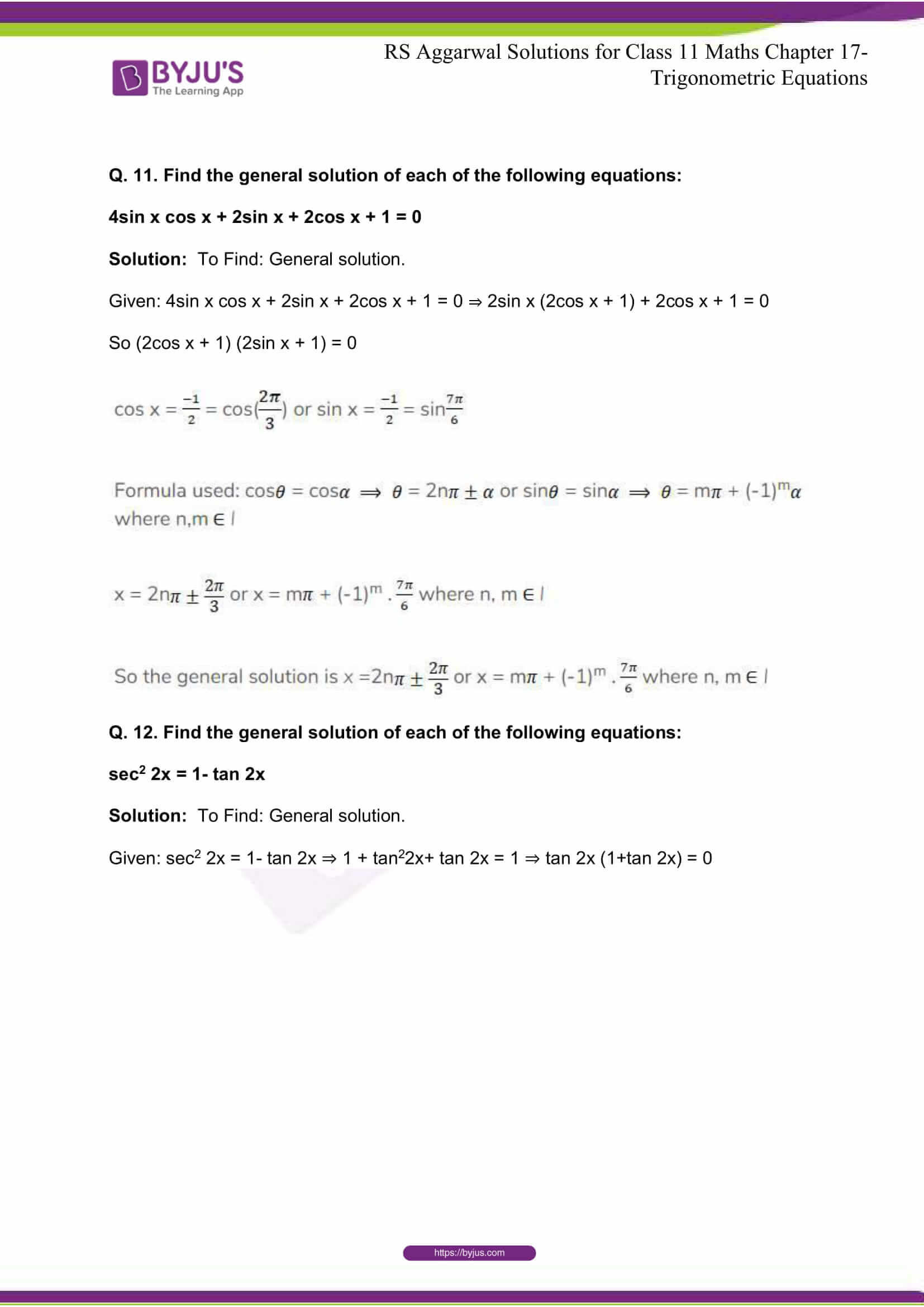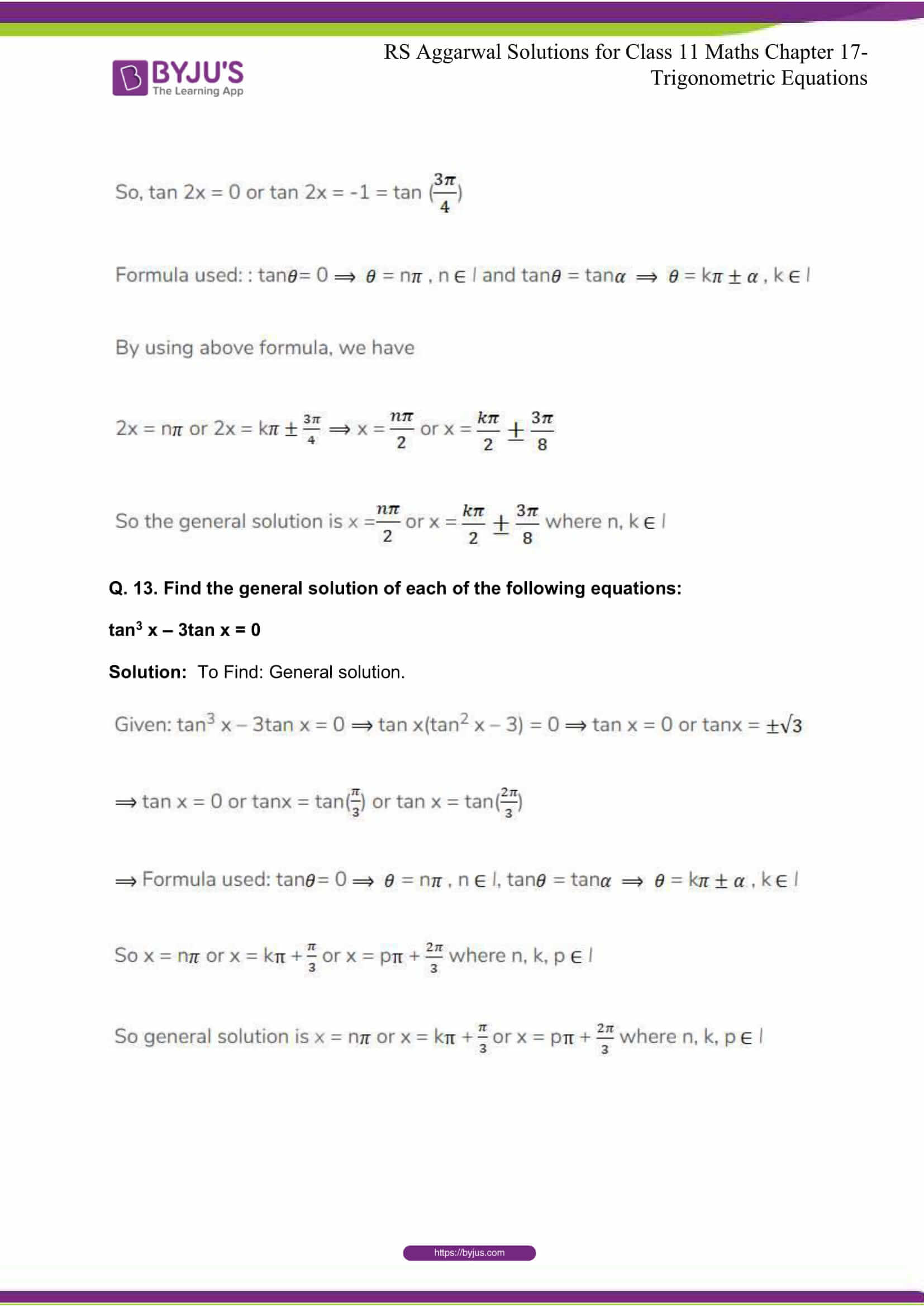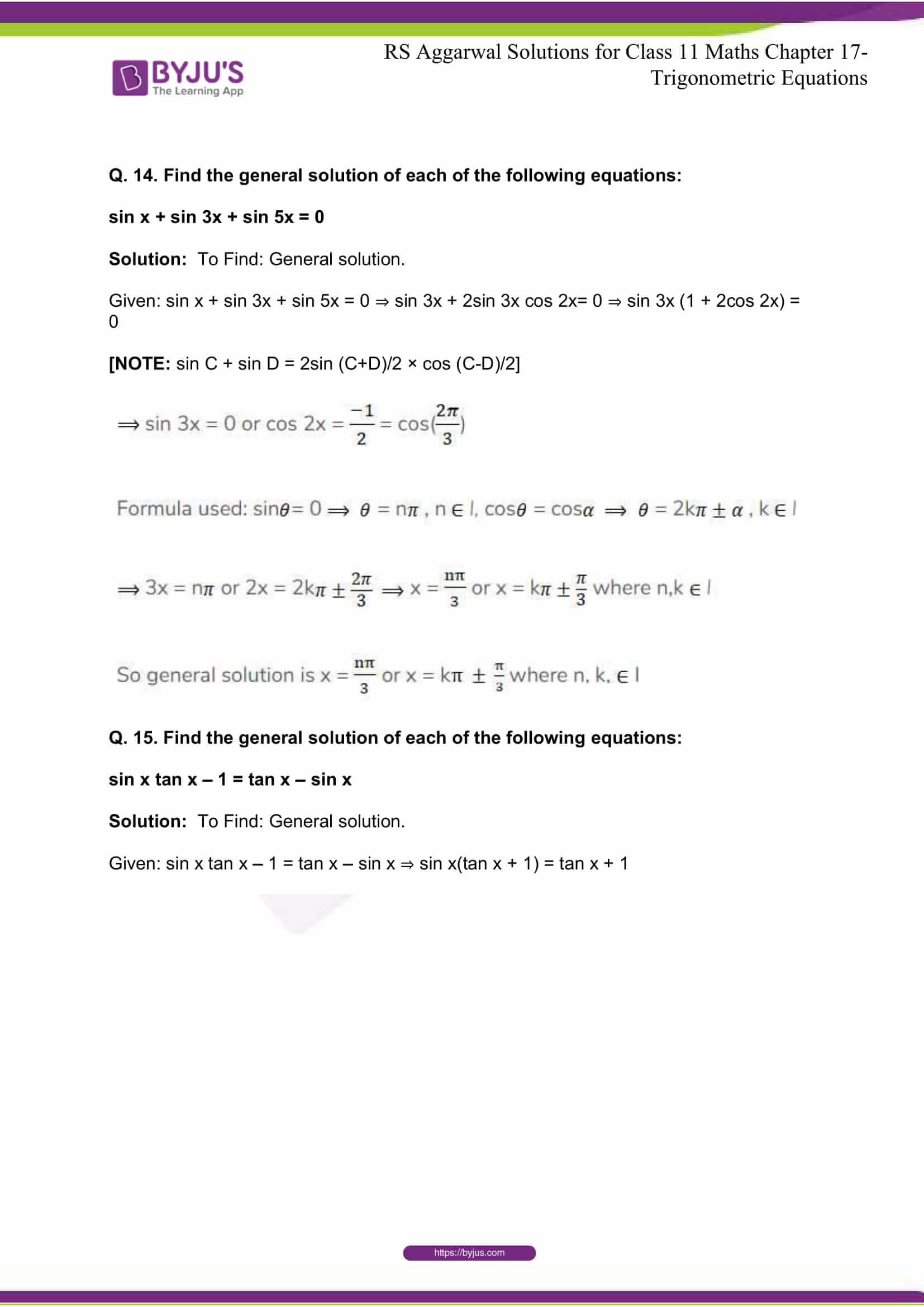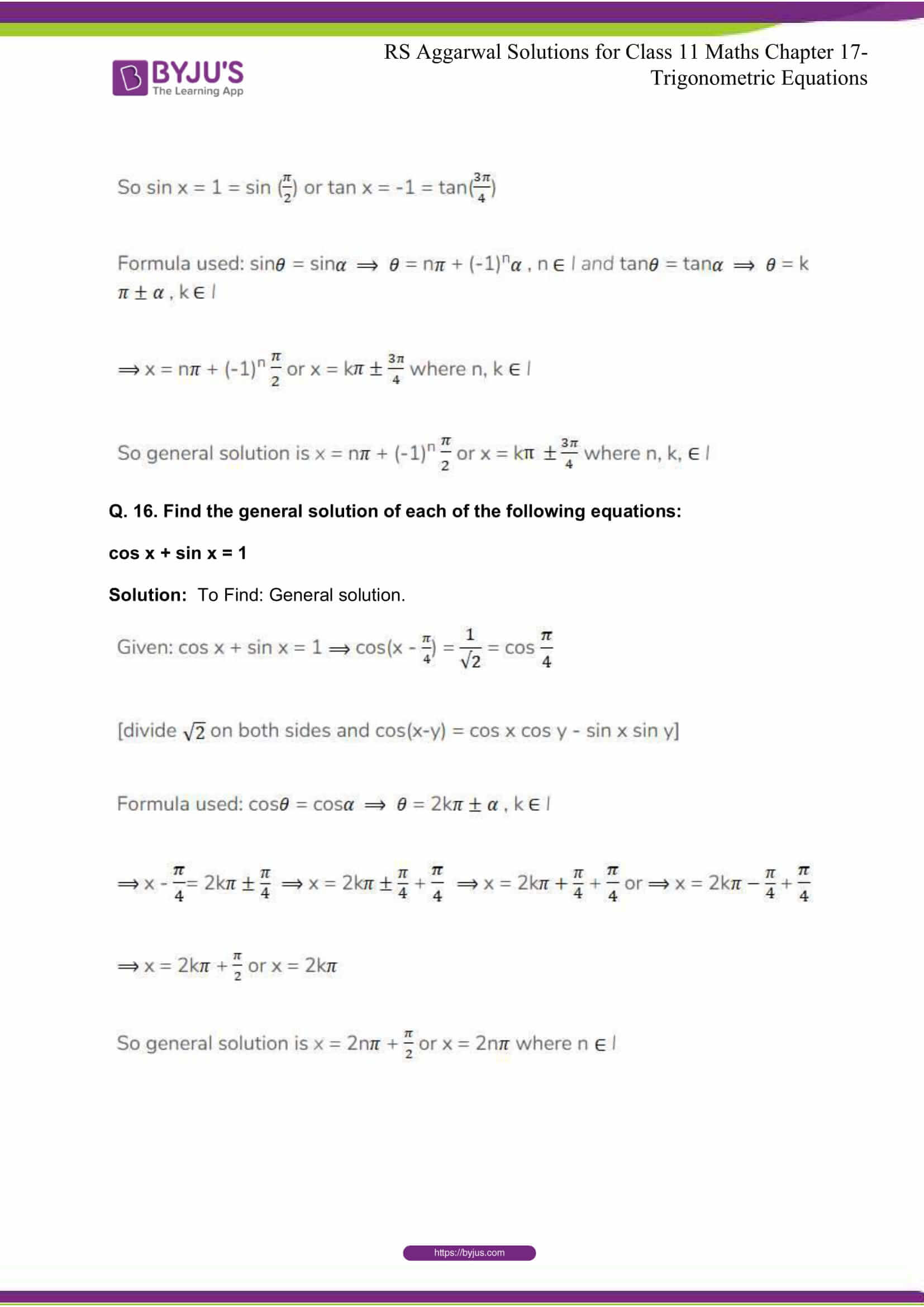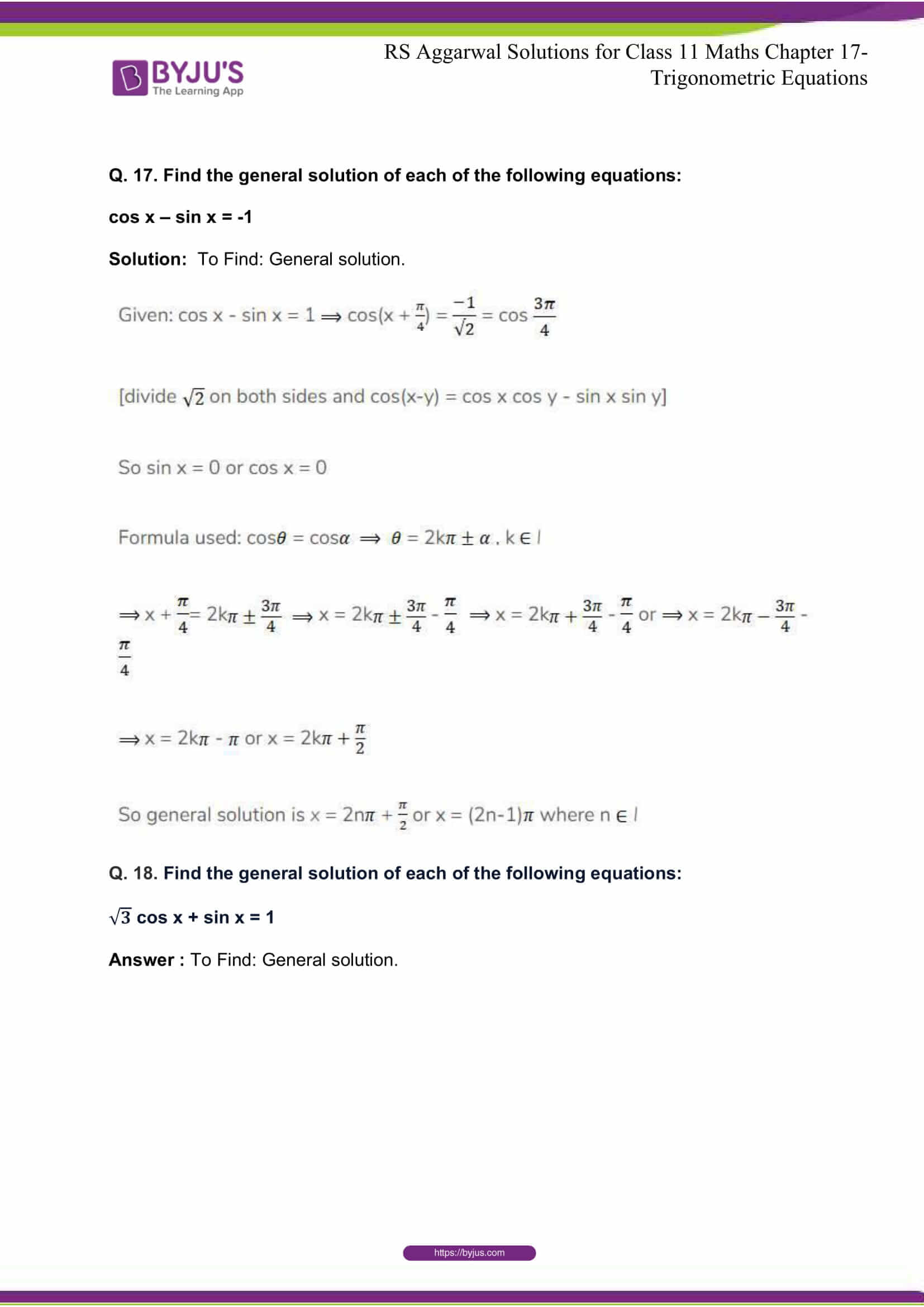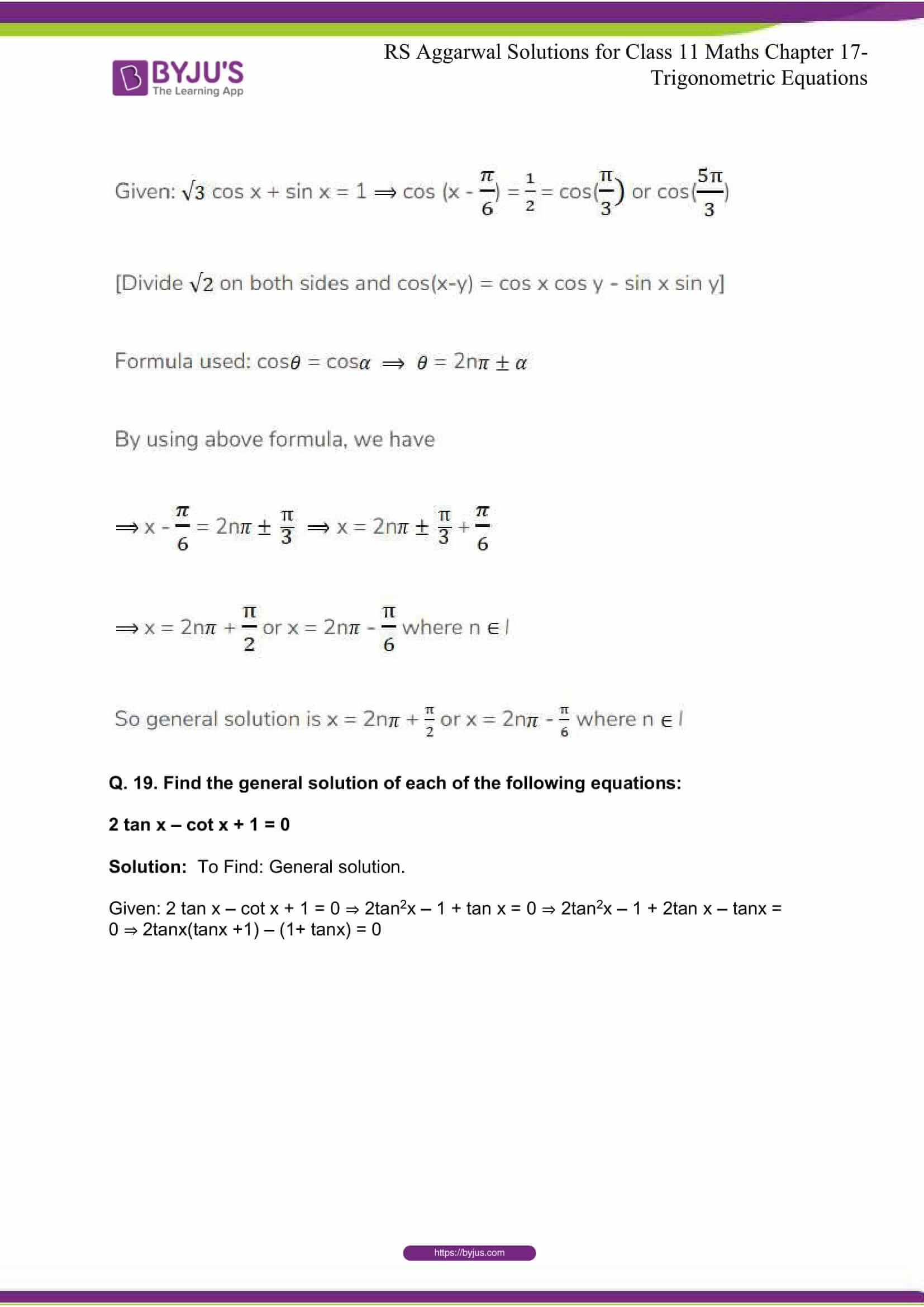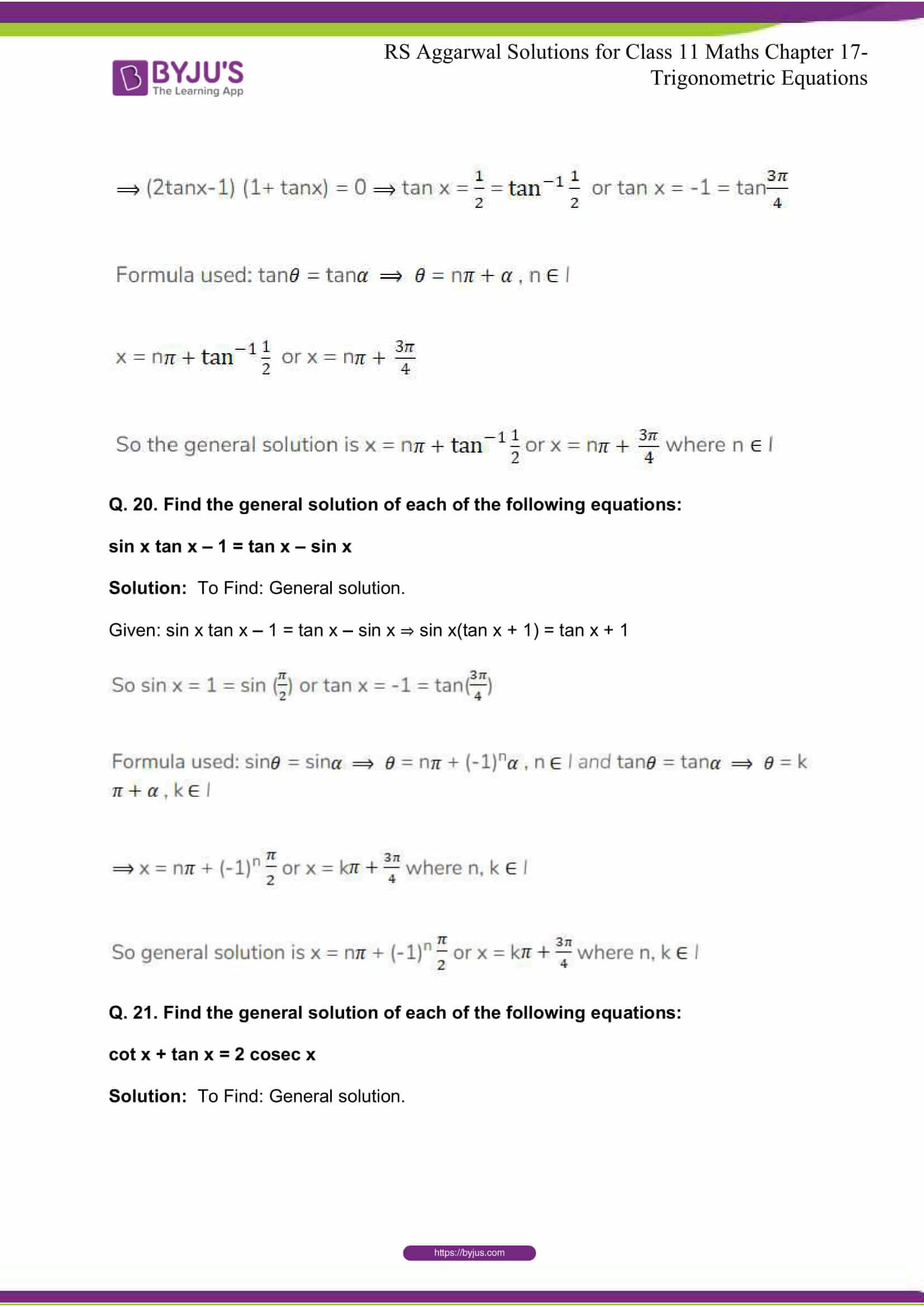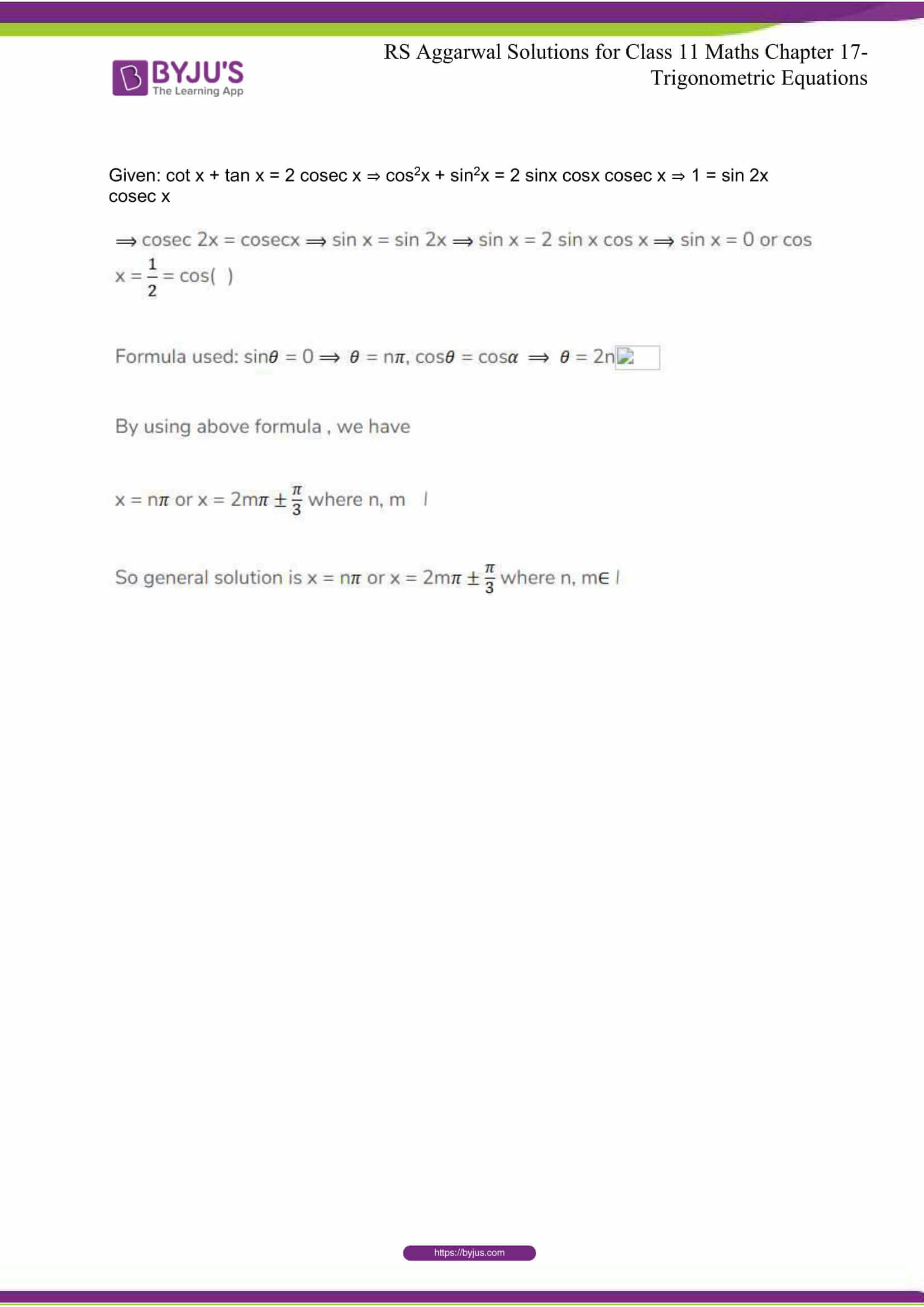## R S Aggarwal Solutions for Class 11 Maths Chapter 17- Trigonometric Equations

The Chapter 17, Trigonometric Equations is composed of 1 exercise and the solutions given here contain answers to all the questions present in the exercise. Let us have a look at some of the topics that are being discussed in this chapter.

17.1 Trigonometric Equations

17.2 Solutions of Trigonometric Equations

17.3 Principal Solutions

17.4 General Solution

17.5 General Solutions of Trigonometric Equations

## R S Aggarwal Solutions for Class 11 Maths Chapter 17- Trigonometric Equations

Trigonometric equations are the equations that includes trigonometric functions. If the equations have such functions and constants alone, then the solution involves finding an unknown, which is an argument to a trigonometric function. Study Chapter 17 of R S Aggarwal textbook to understand more about Trigonometric Equations. Learn the R S Aggarwal Solutions for Class 11 effectively to come out with flying colours in the examinations.